# Concise Selina Solutions for Class 9 Maths Chapter 6- Simultaneous Equations

Selina solutions for Class 9 Maths chapter 6 Simultaneous Equations are provided here. Class 9 is an important phase of a student’s life as the concepts which are taught in Class 9 are to be continued in Class 10. To score good marks in Class 9 Mathematics examination, it is advised to solve questions provided in each exercise of all the chapters in the Selina book. These Selina solutions for Class 9 Maths help the students in understanding the concepts given in a better way. Download pdf of Class 9 Maths chapter 6 Selina Solutions from the given links.

### Download PDF of Selina Solutions for Class 9 Maths Chapter 6- Simultaneous Equations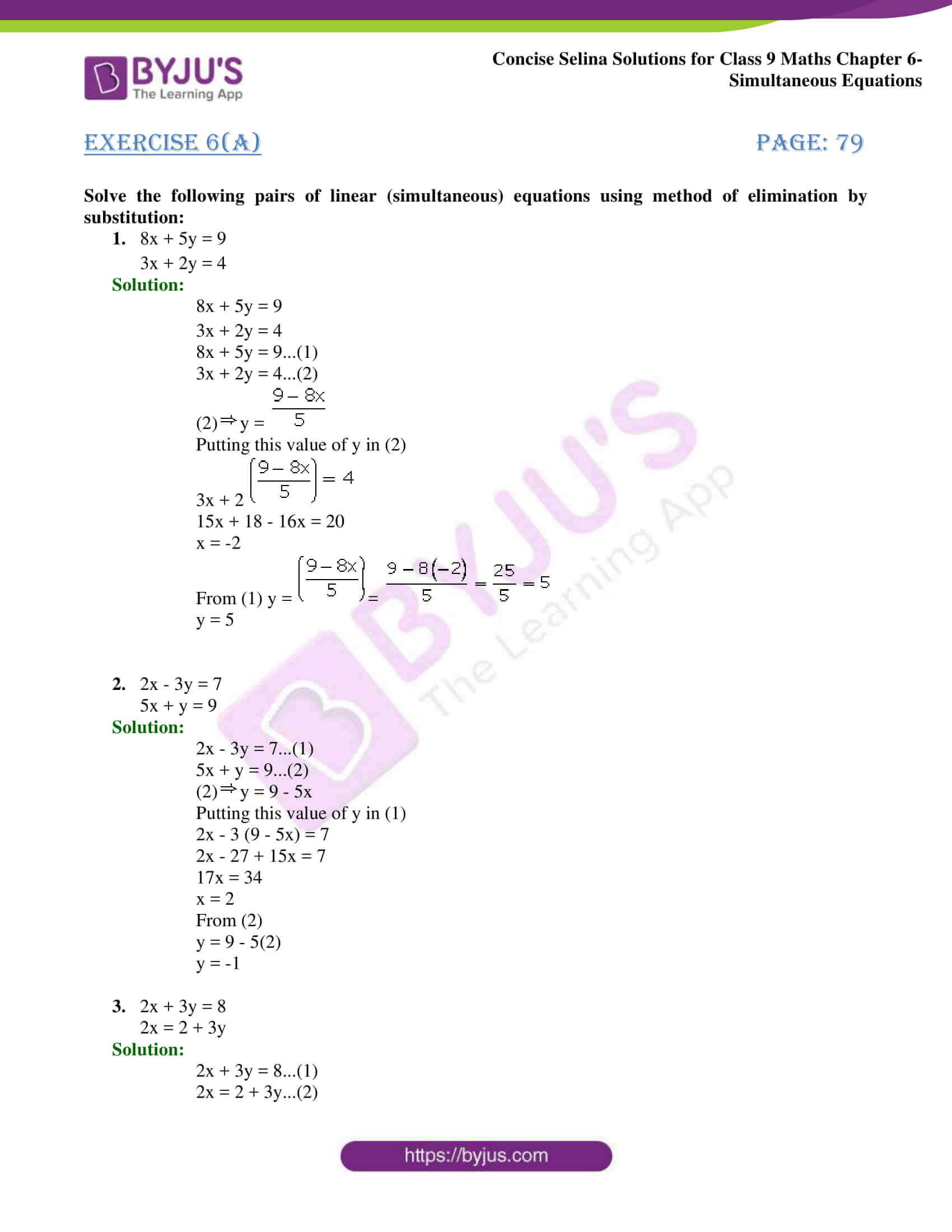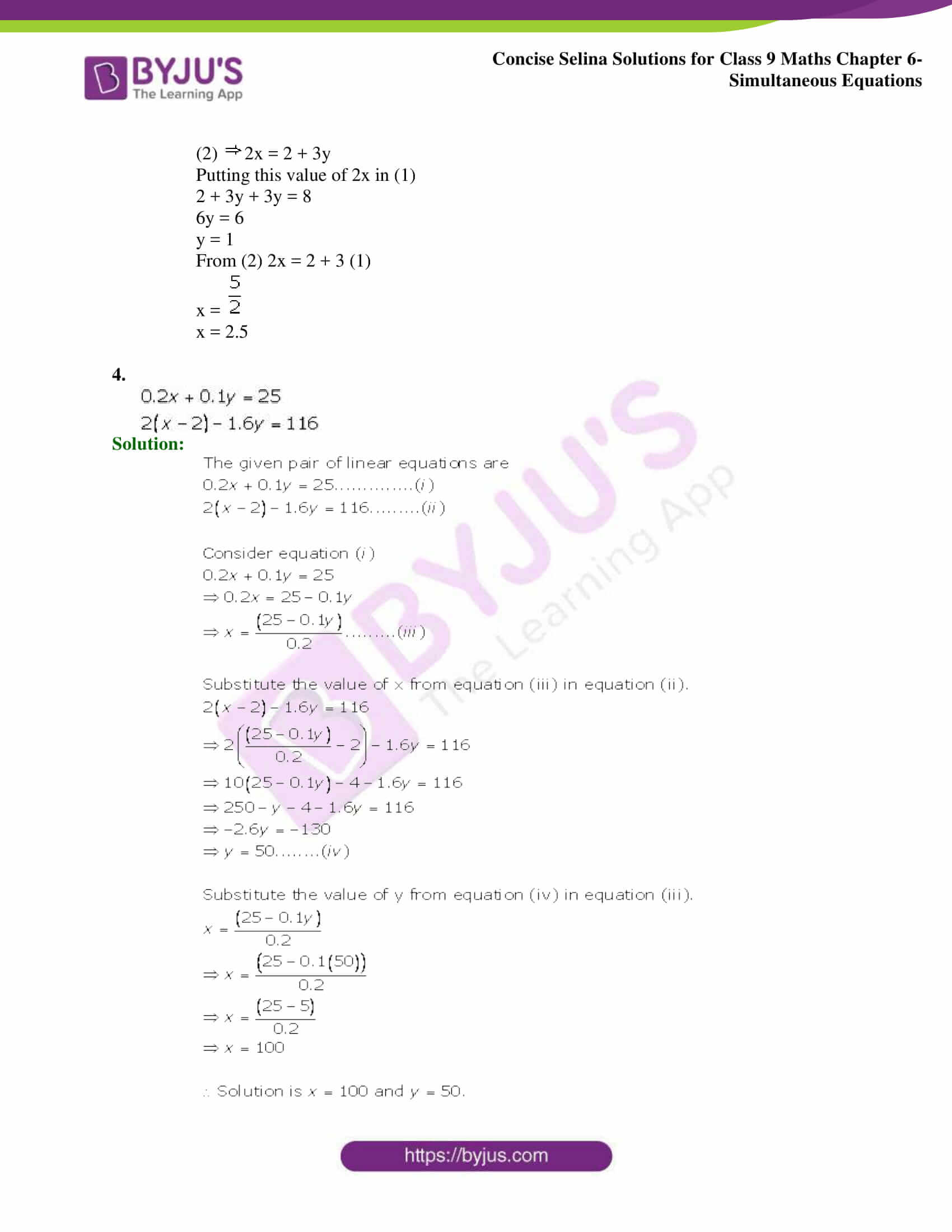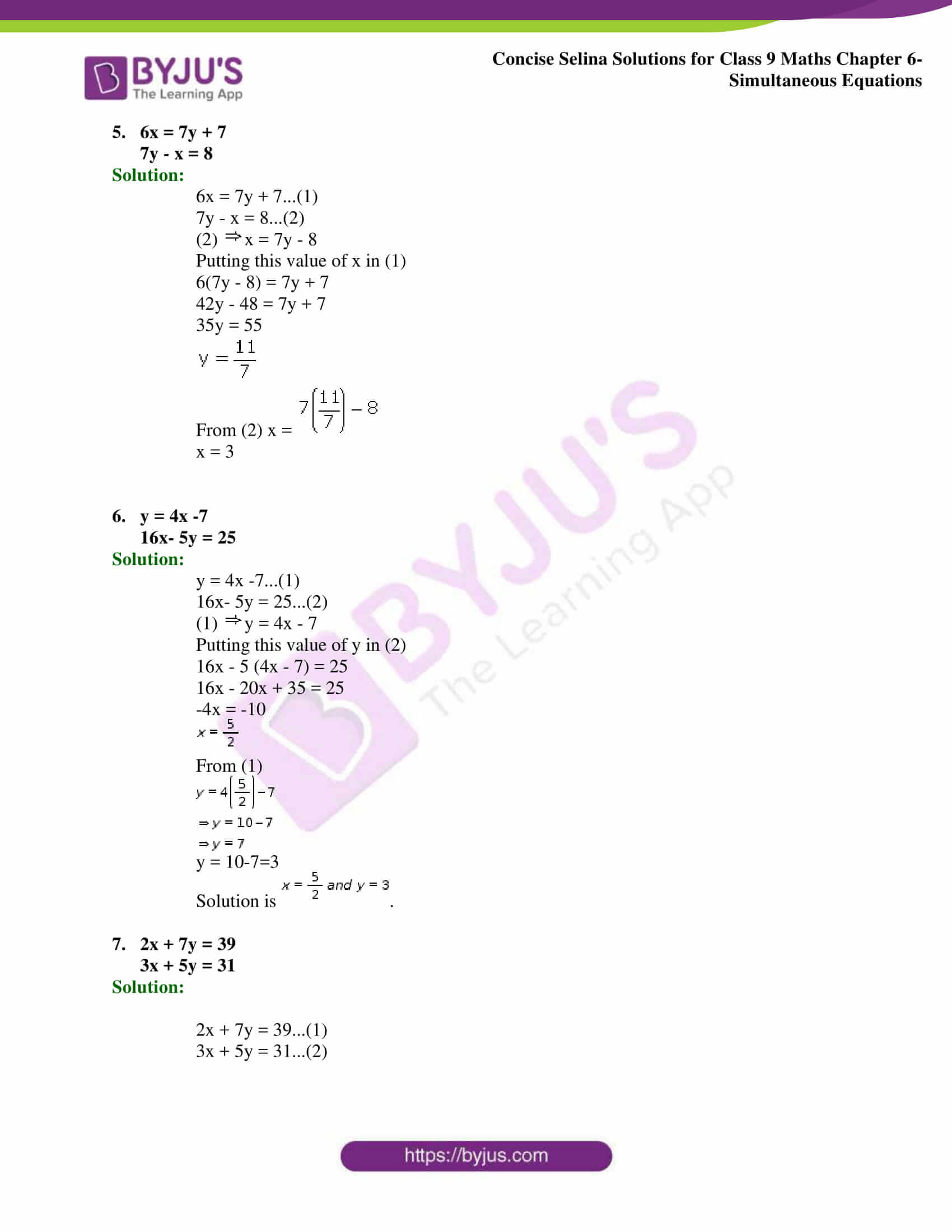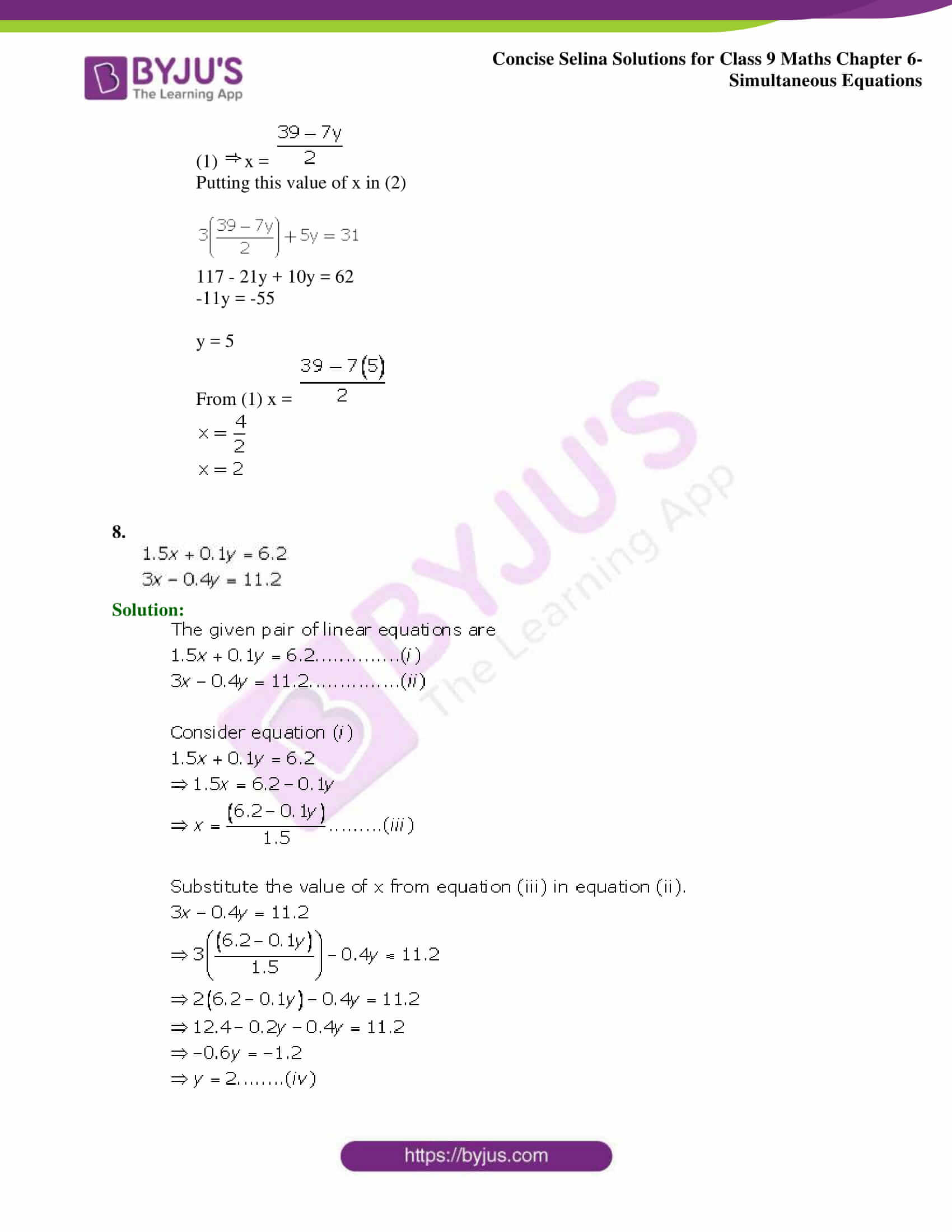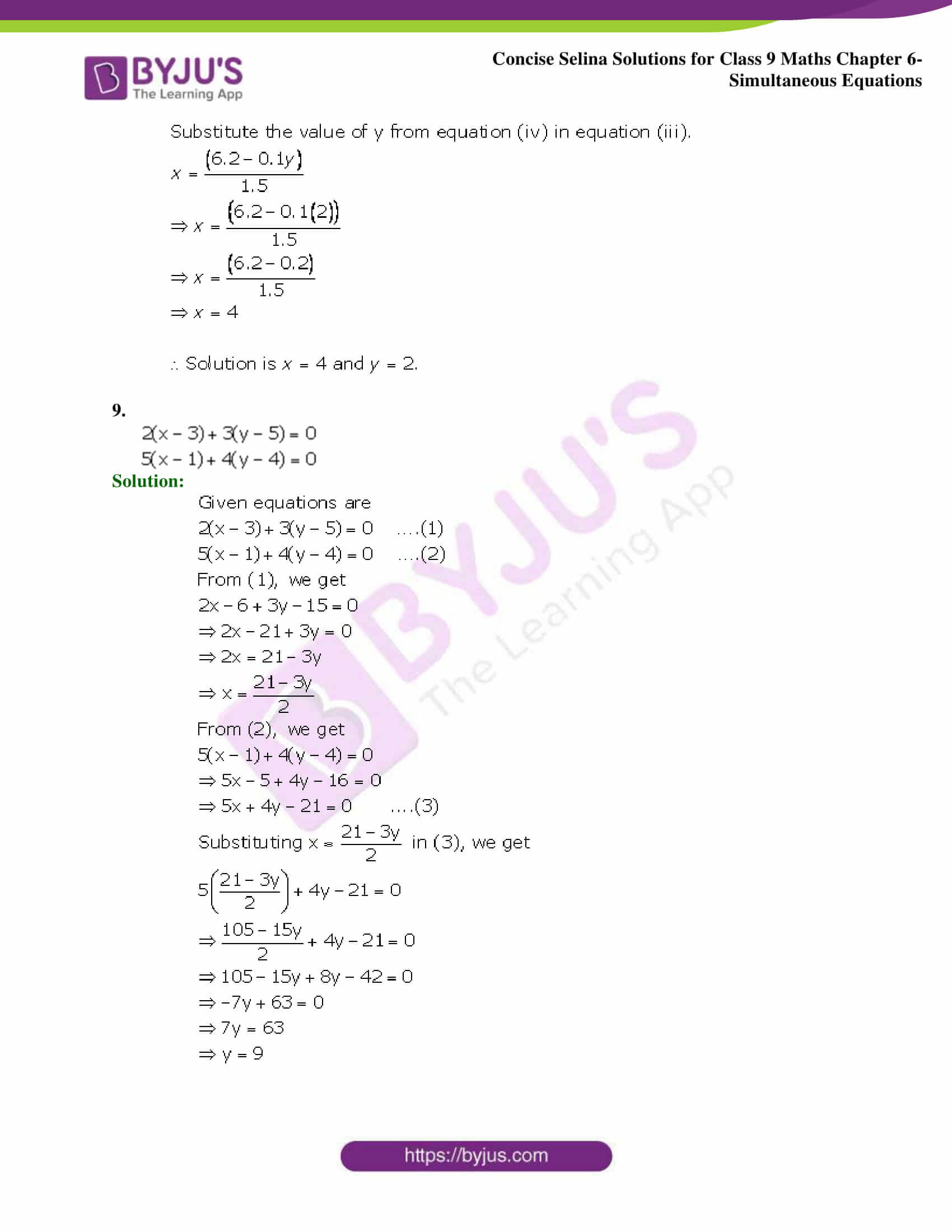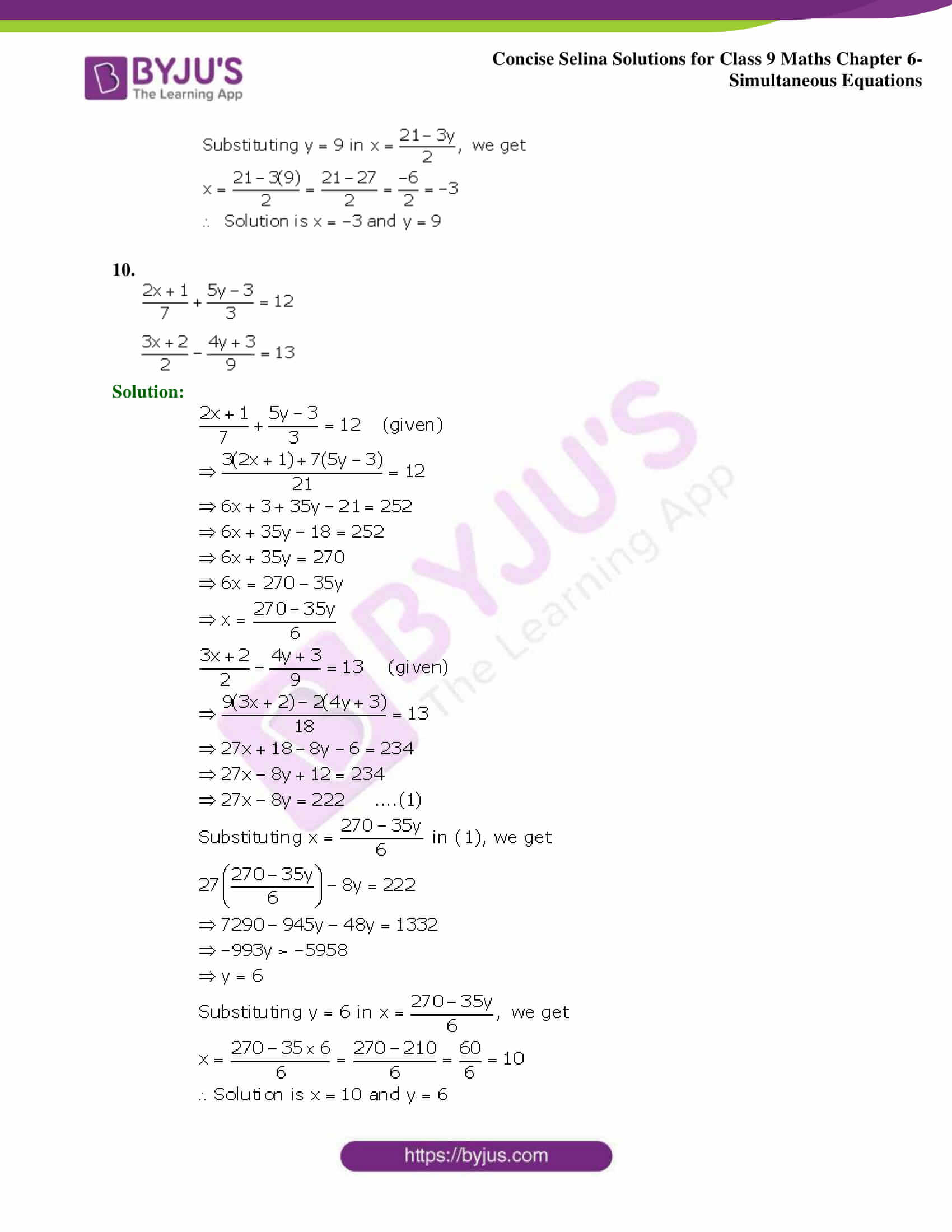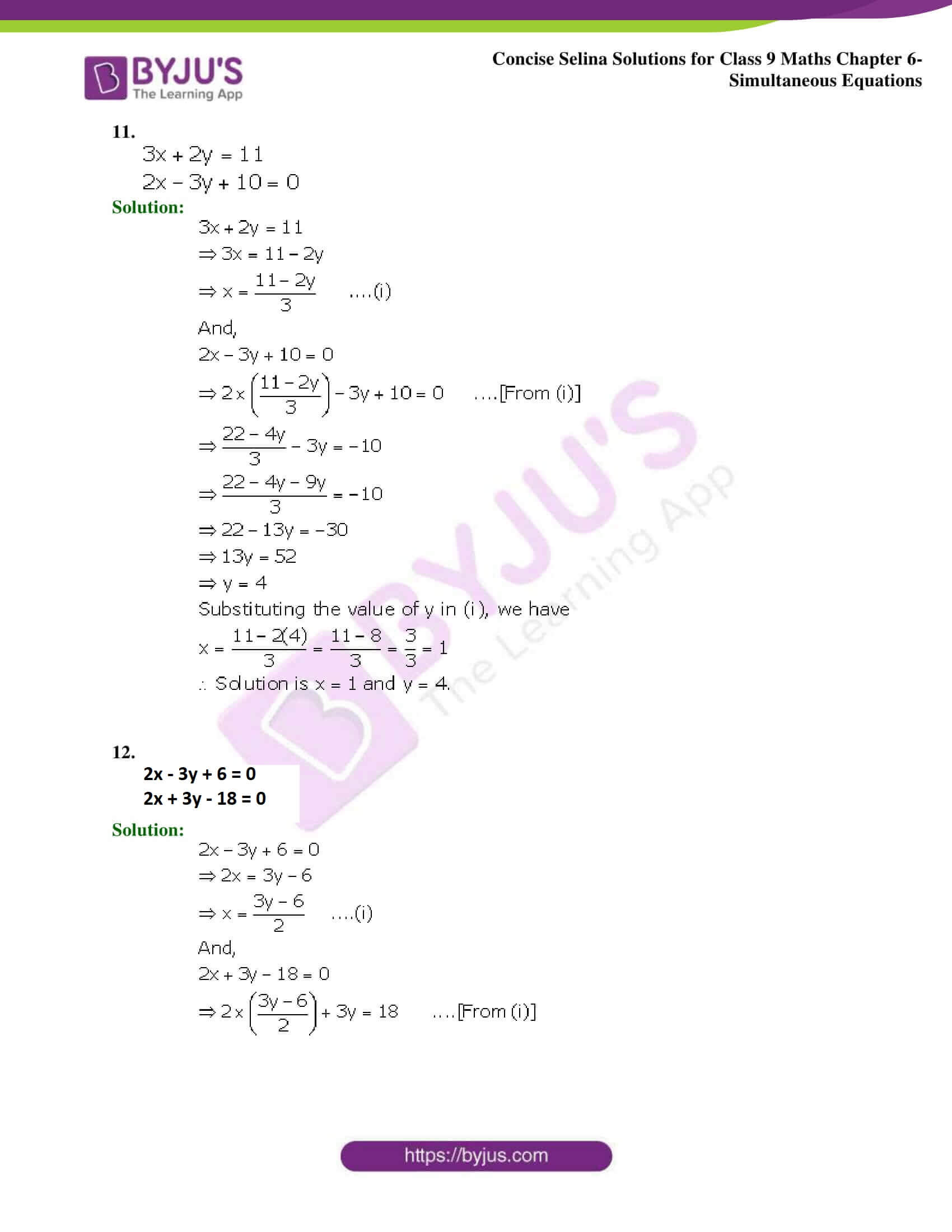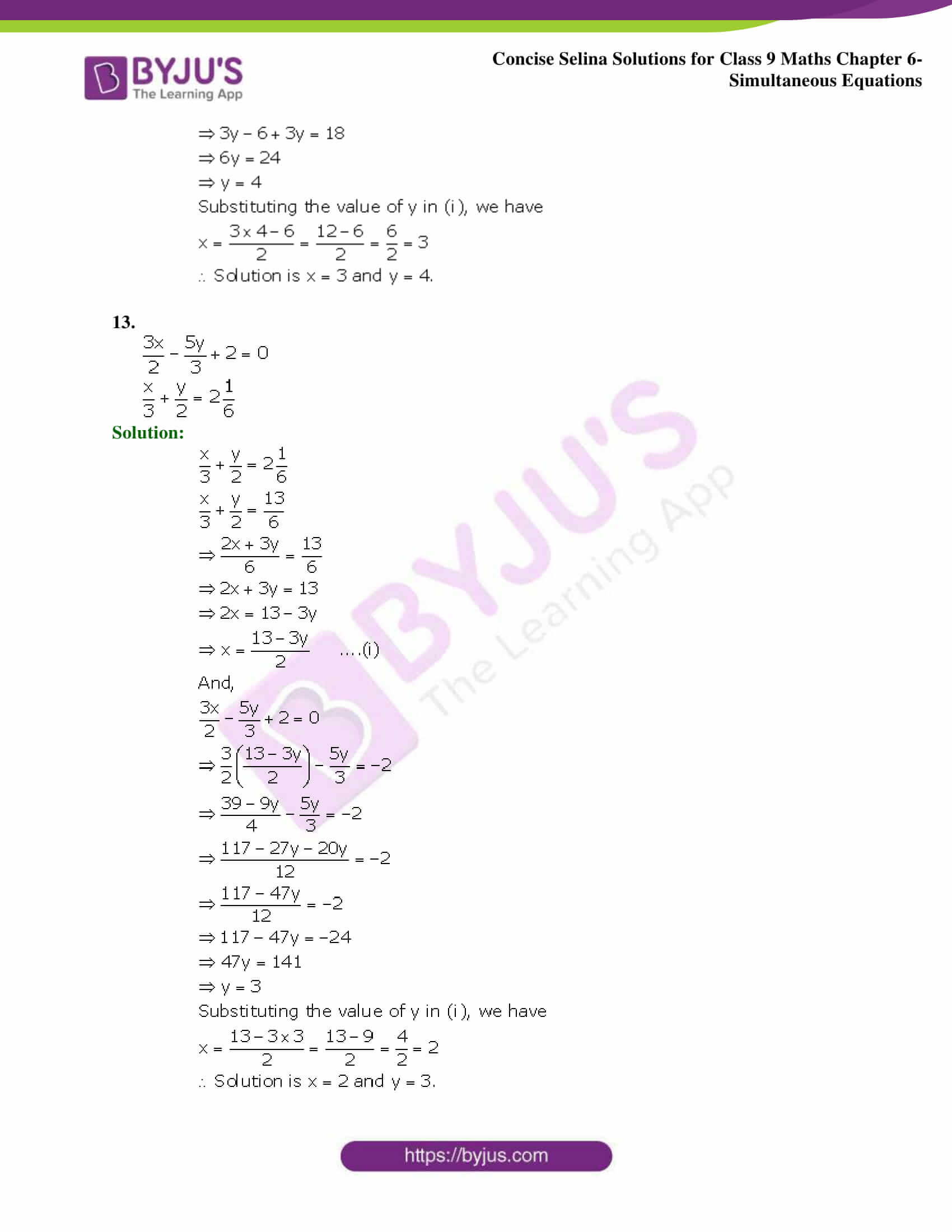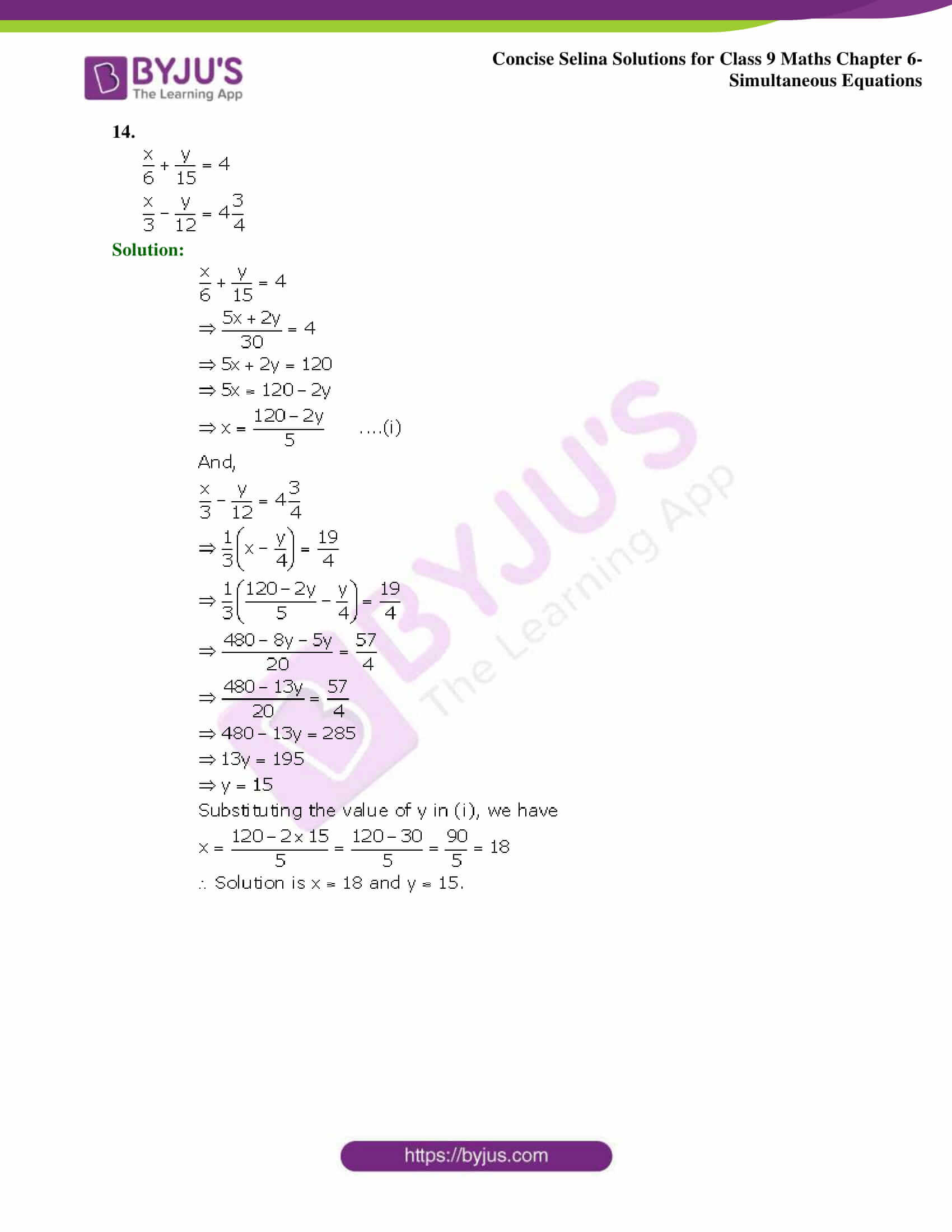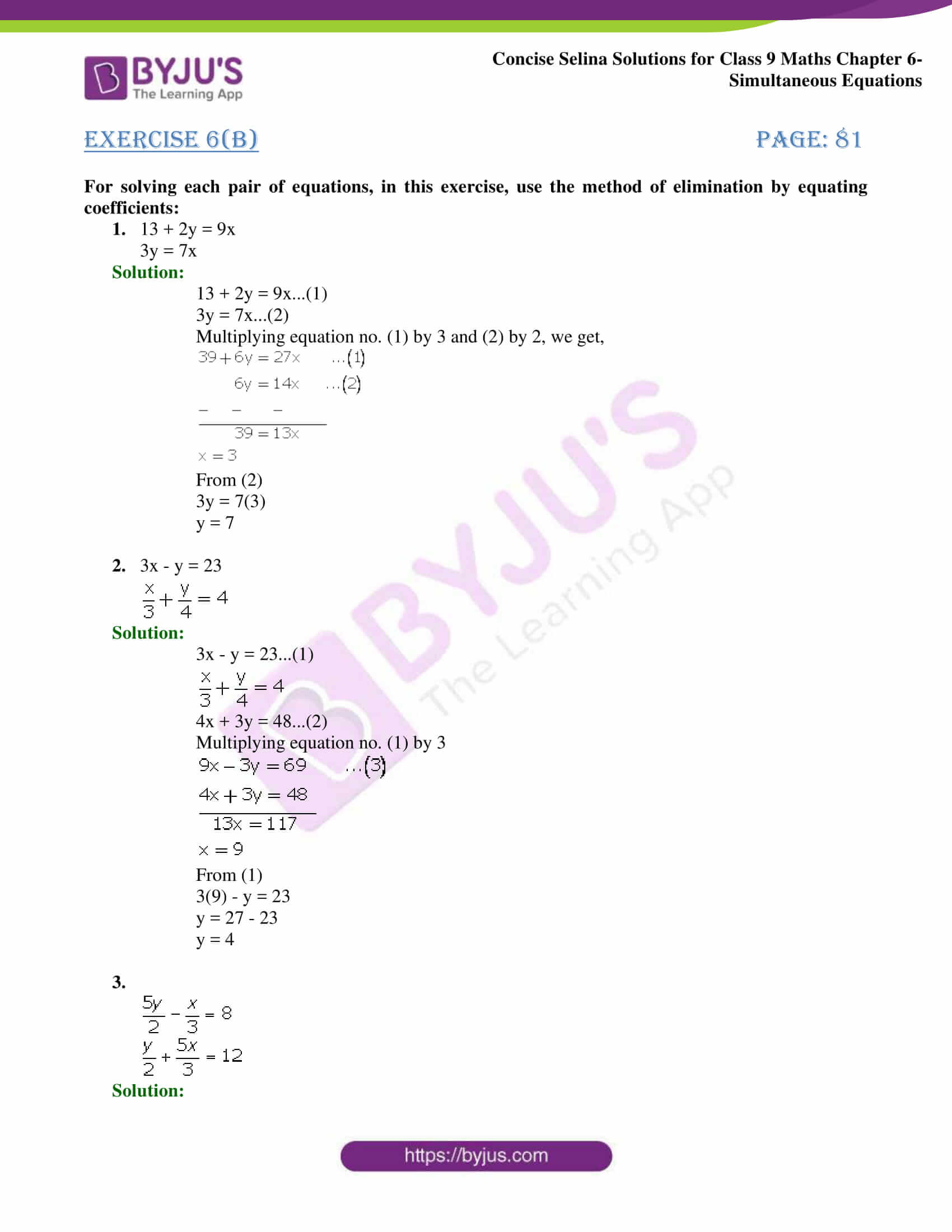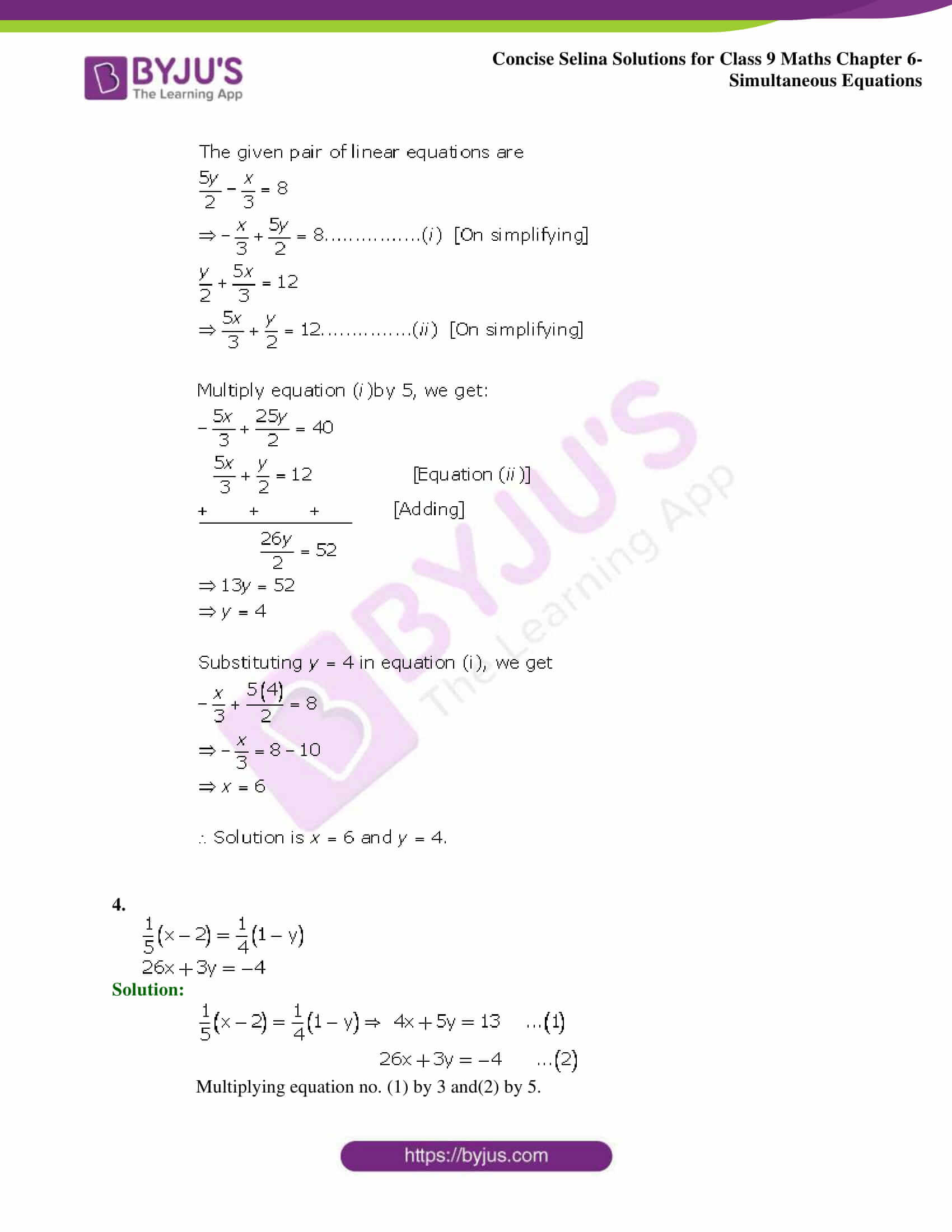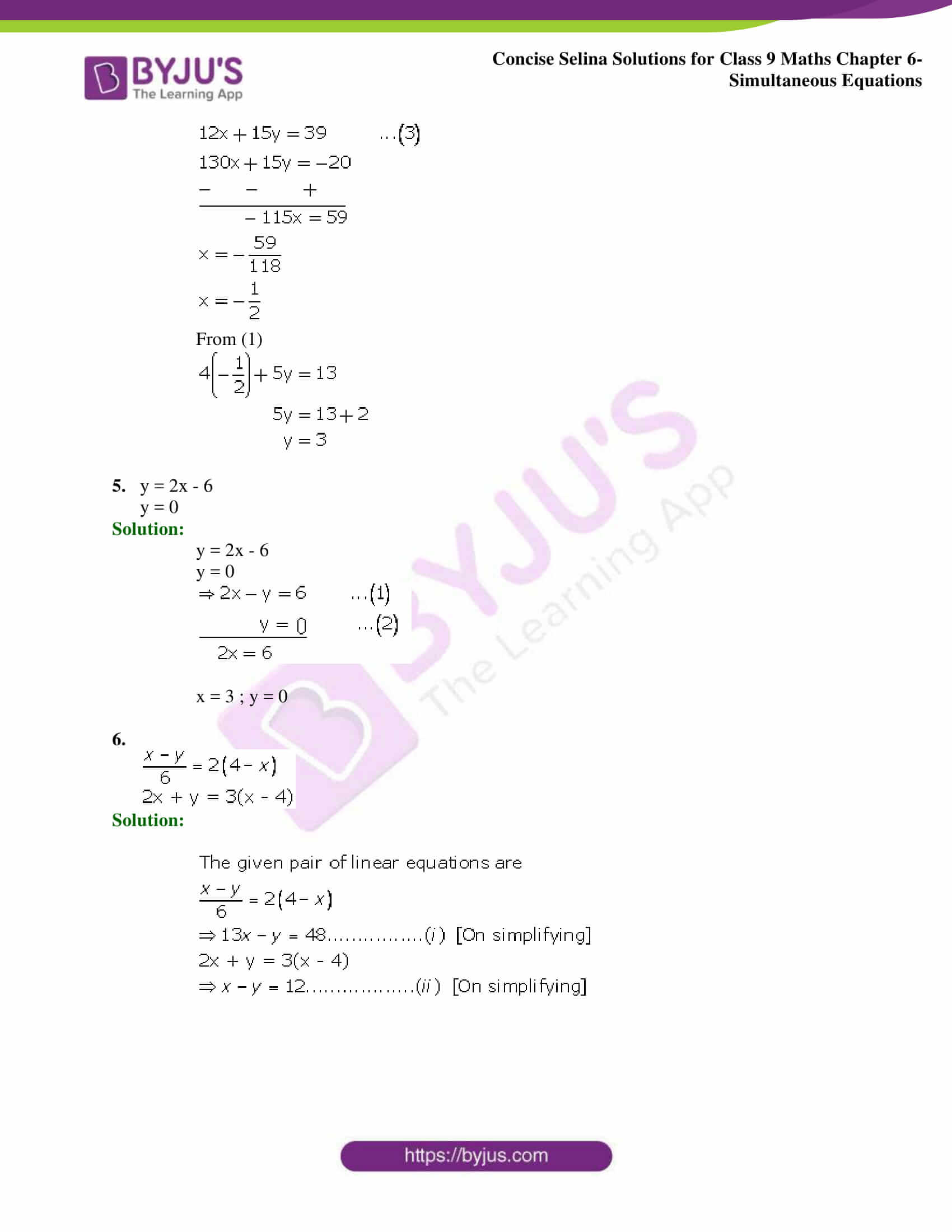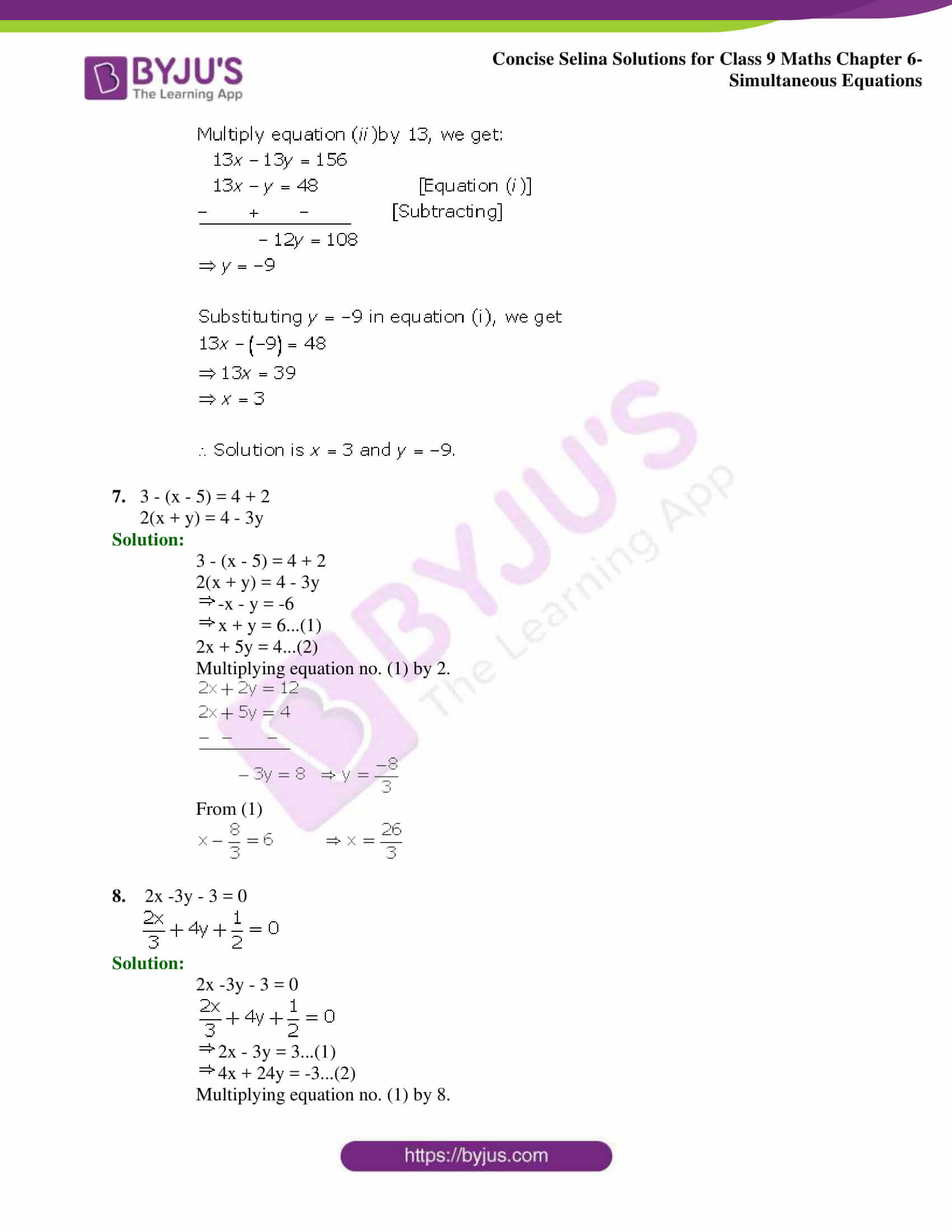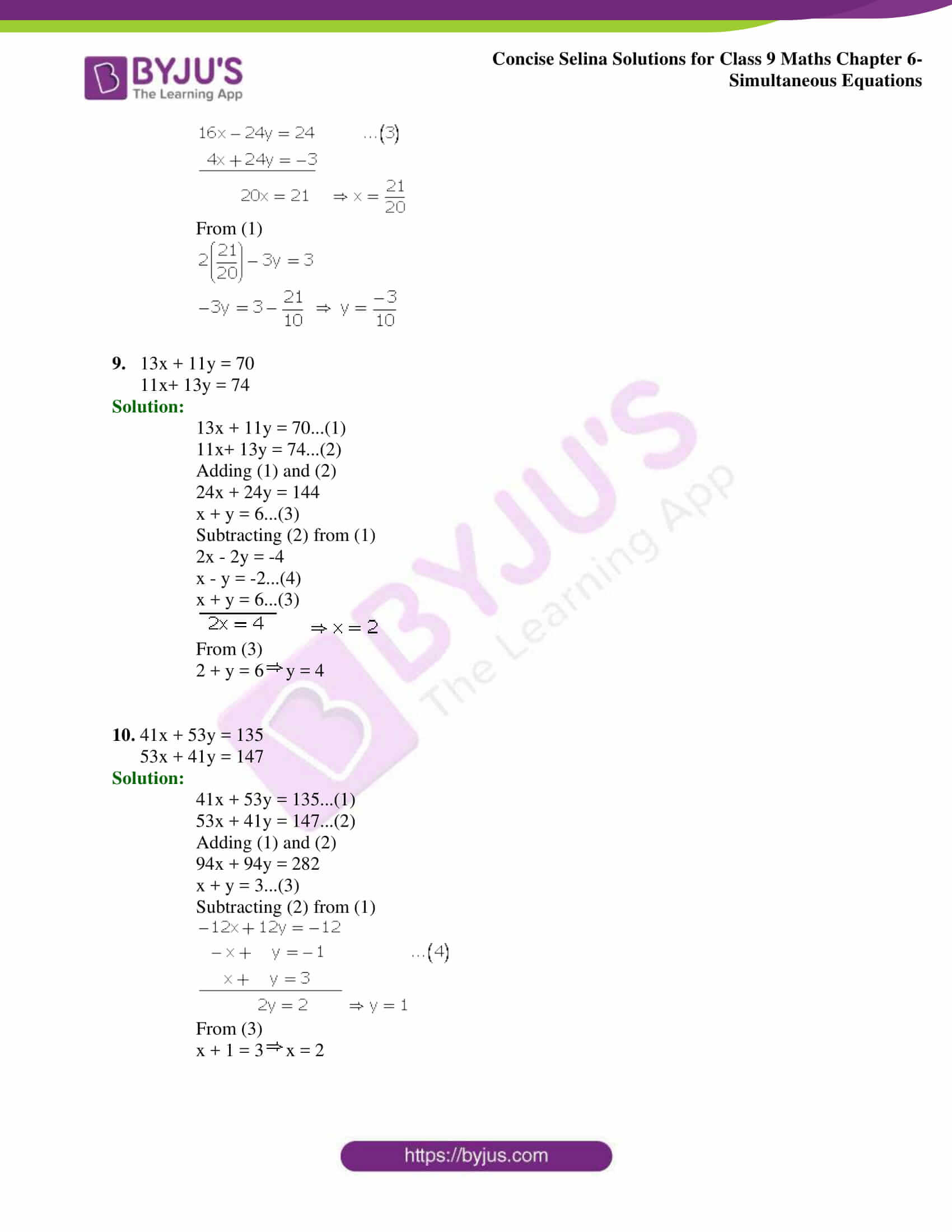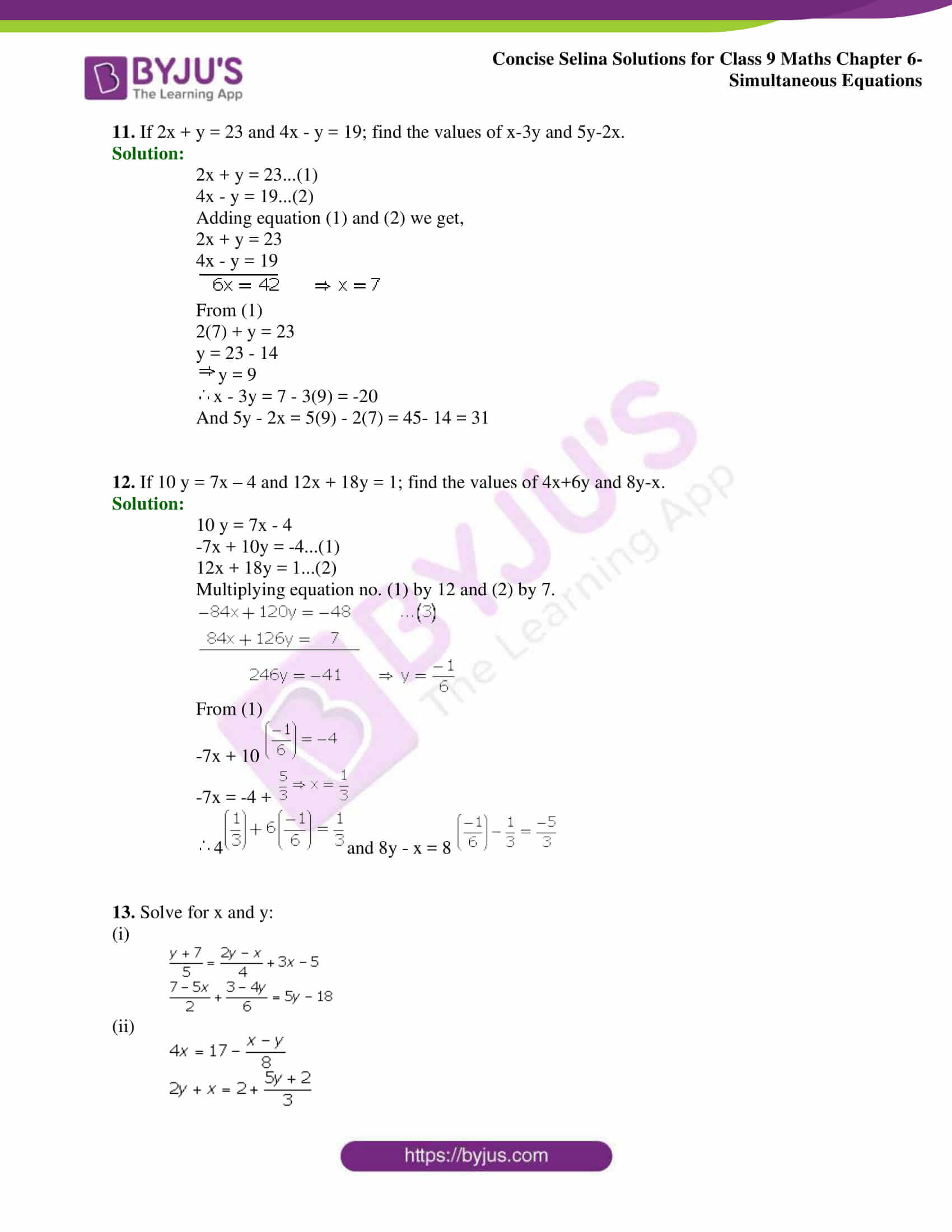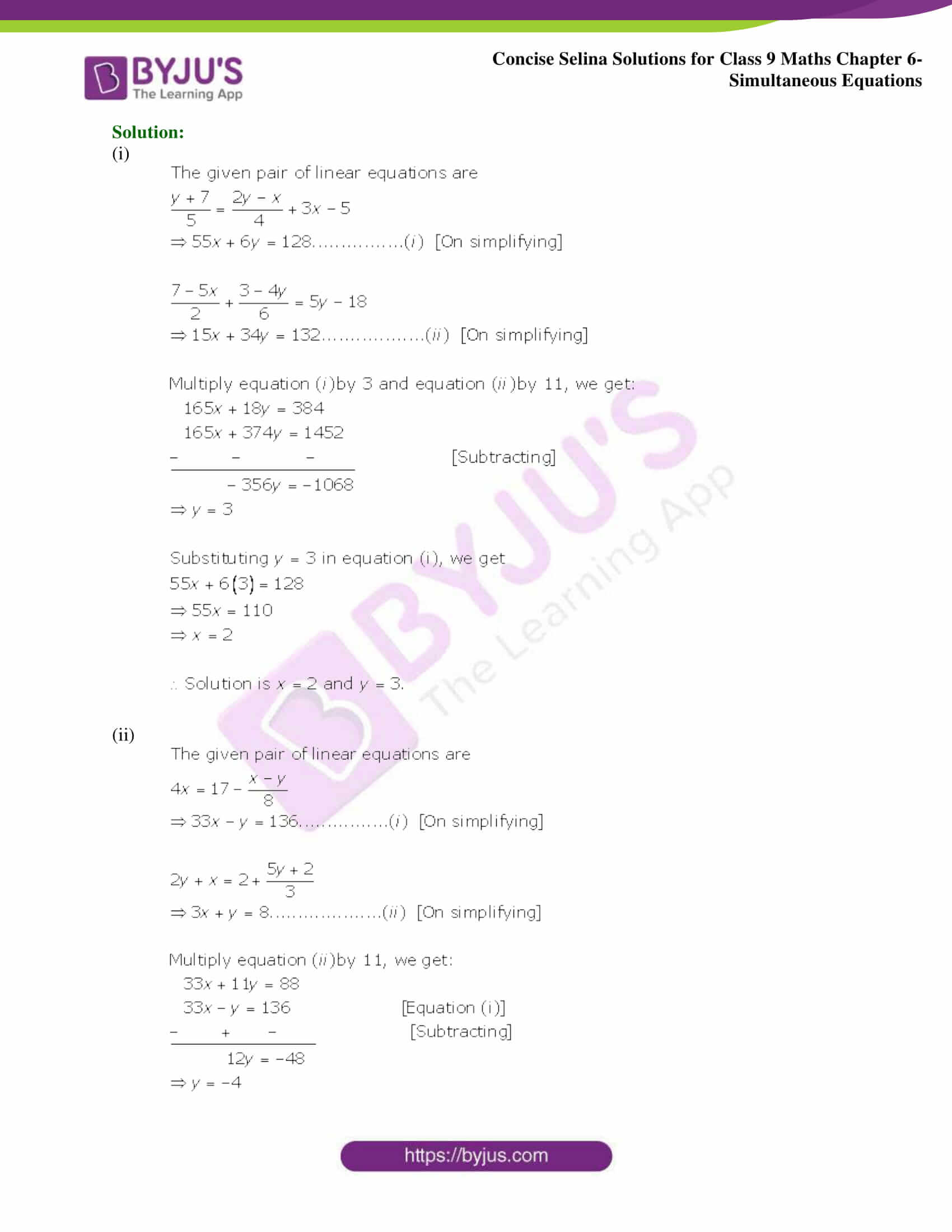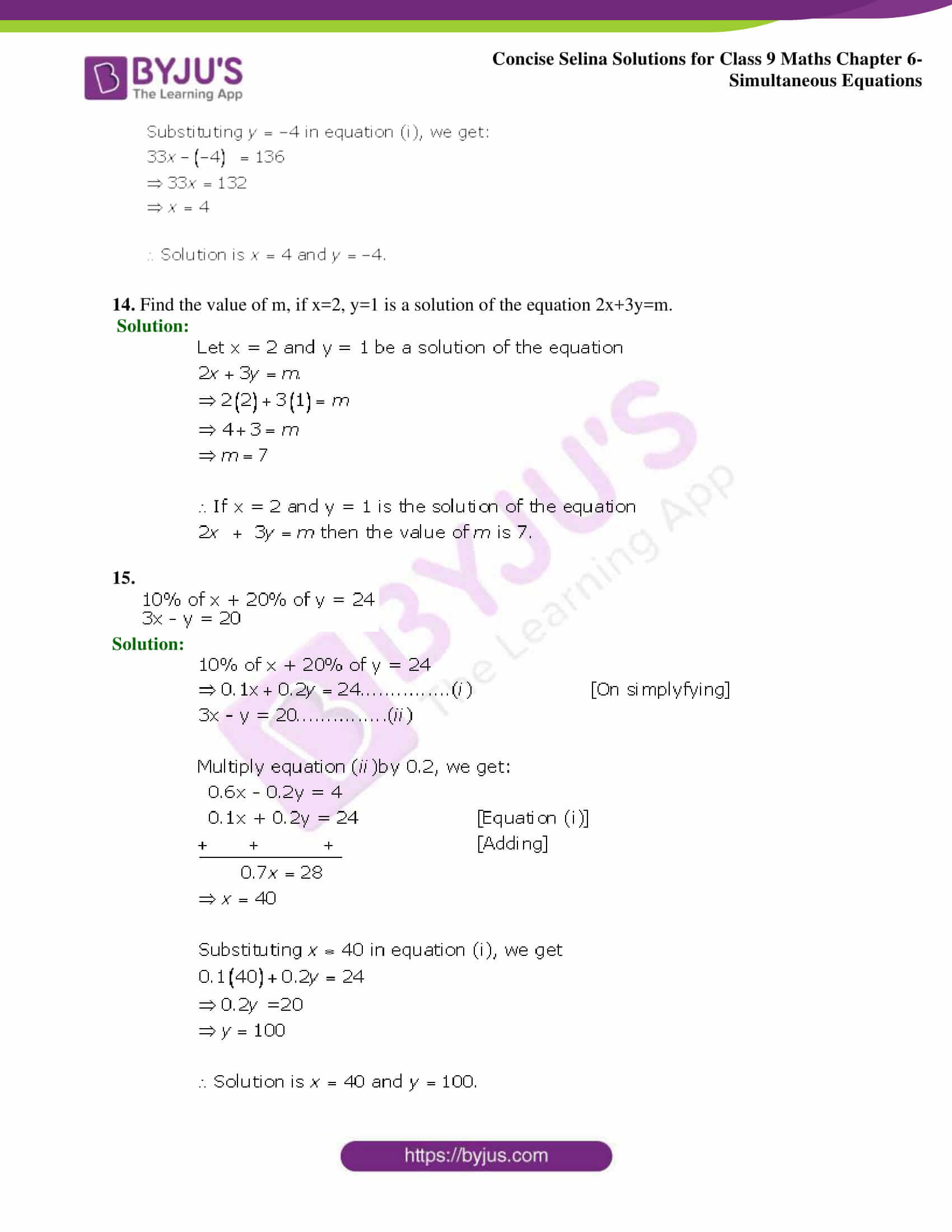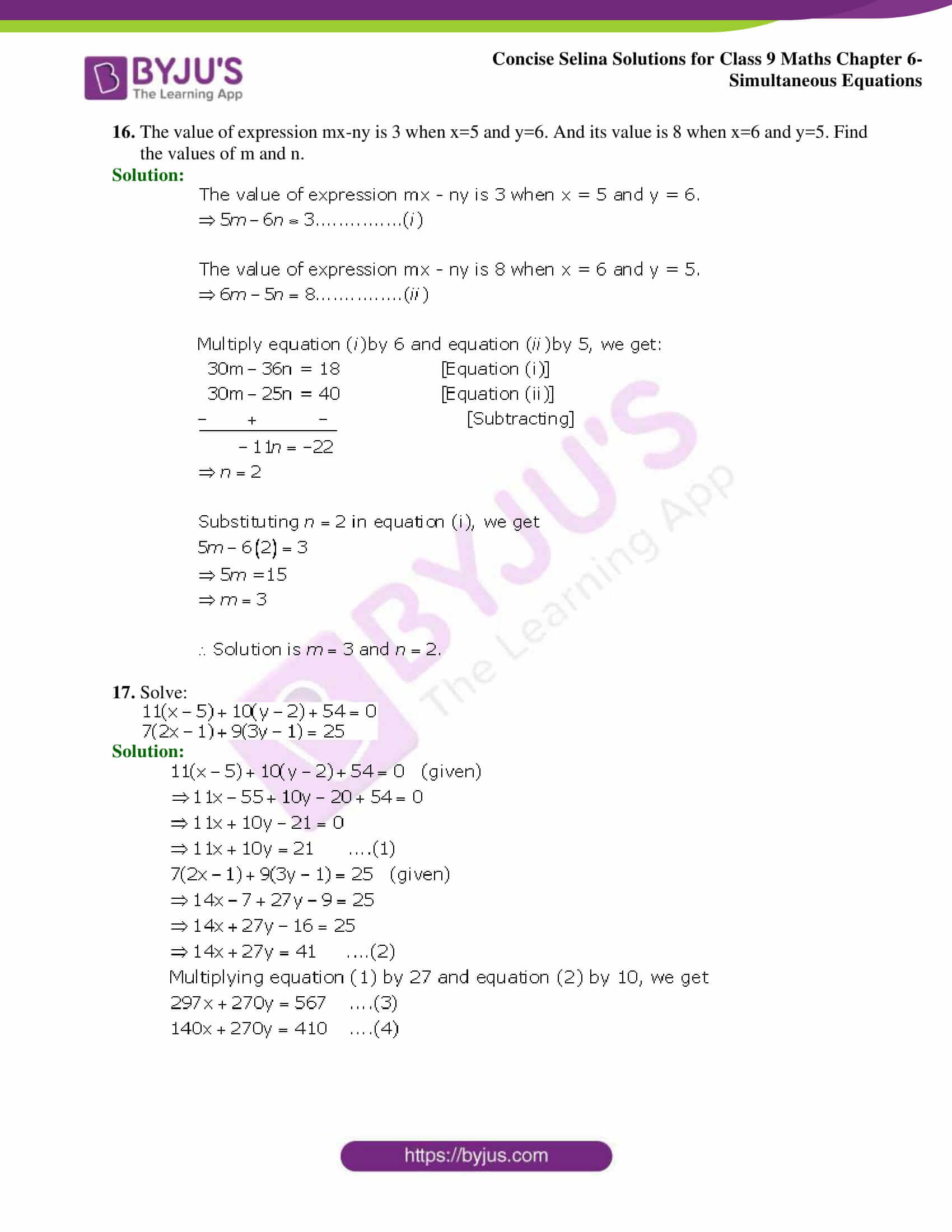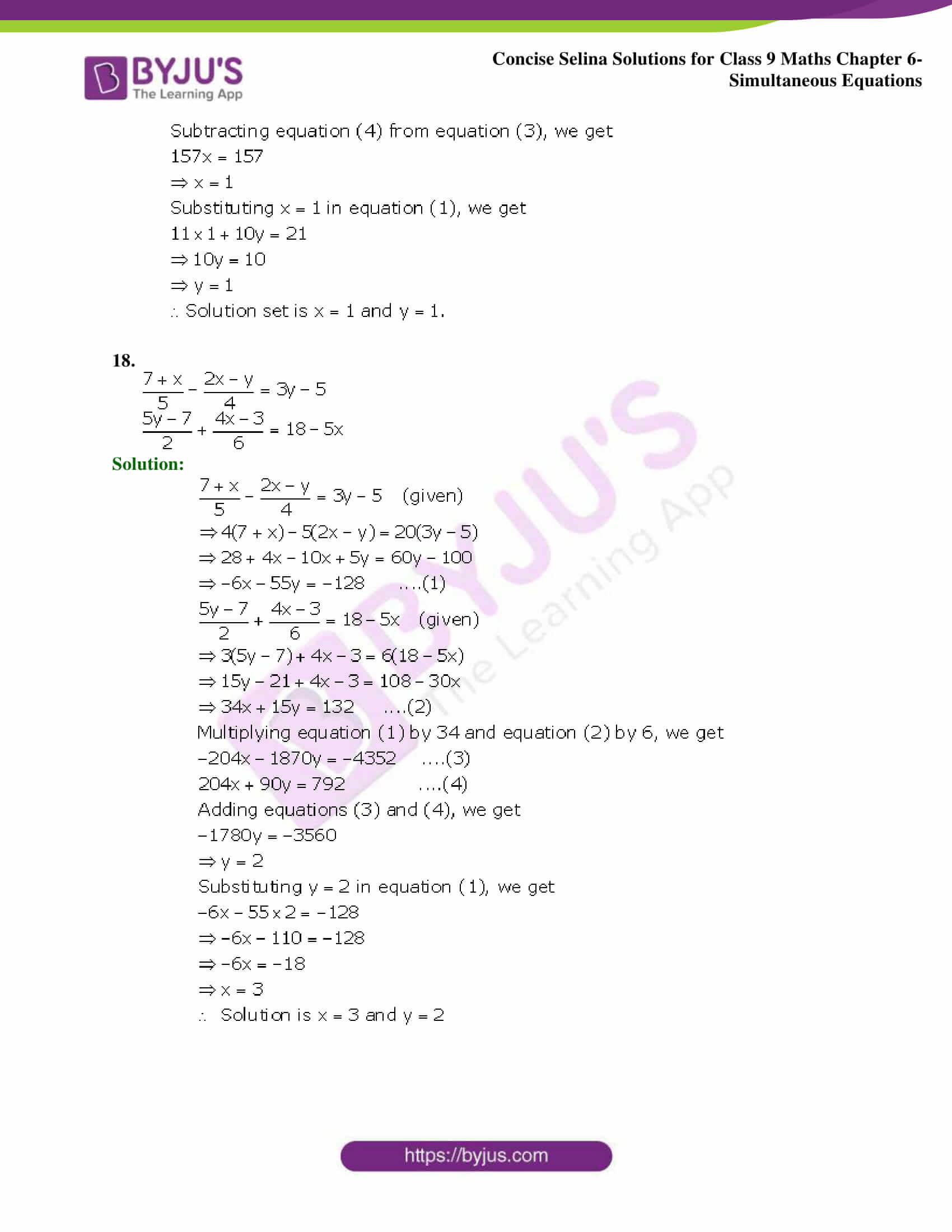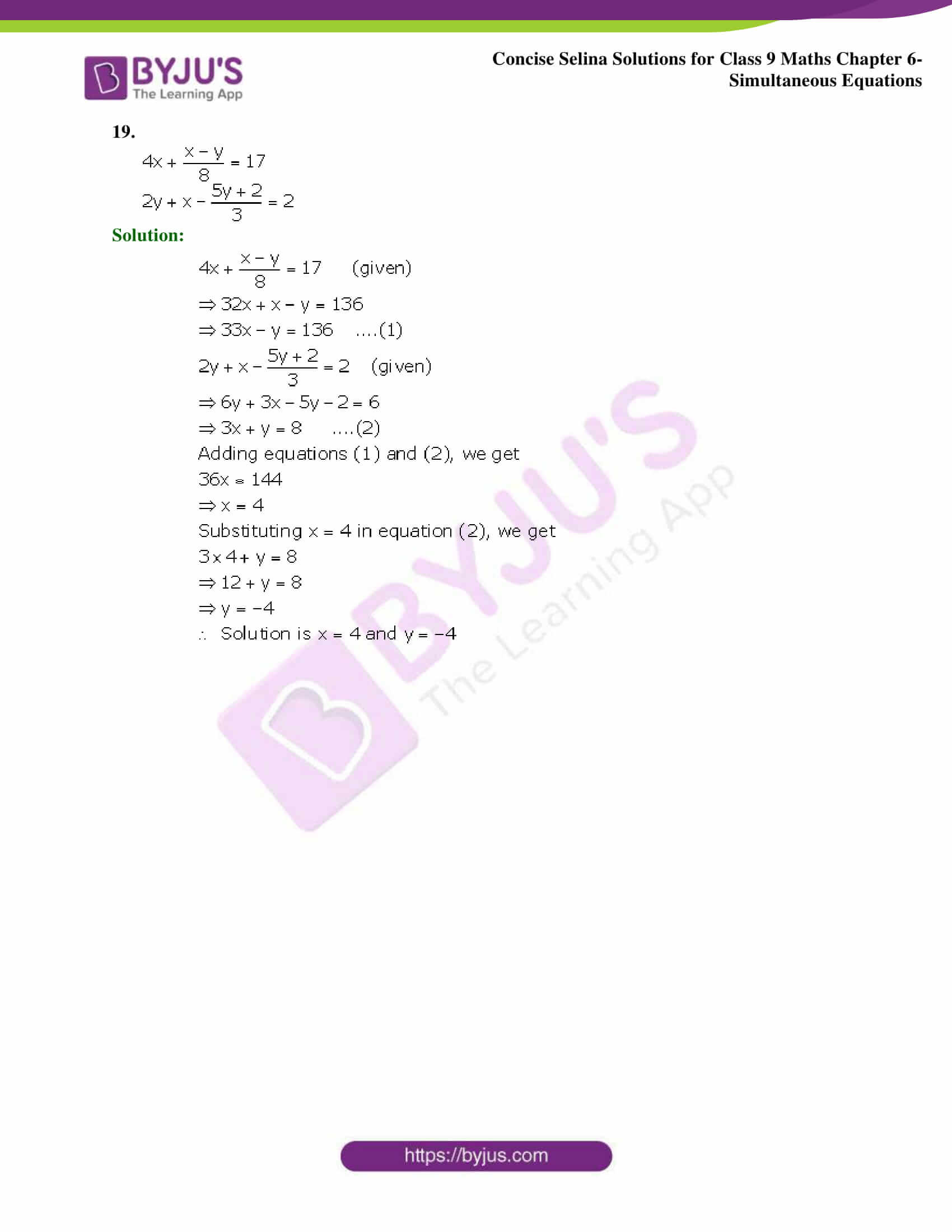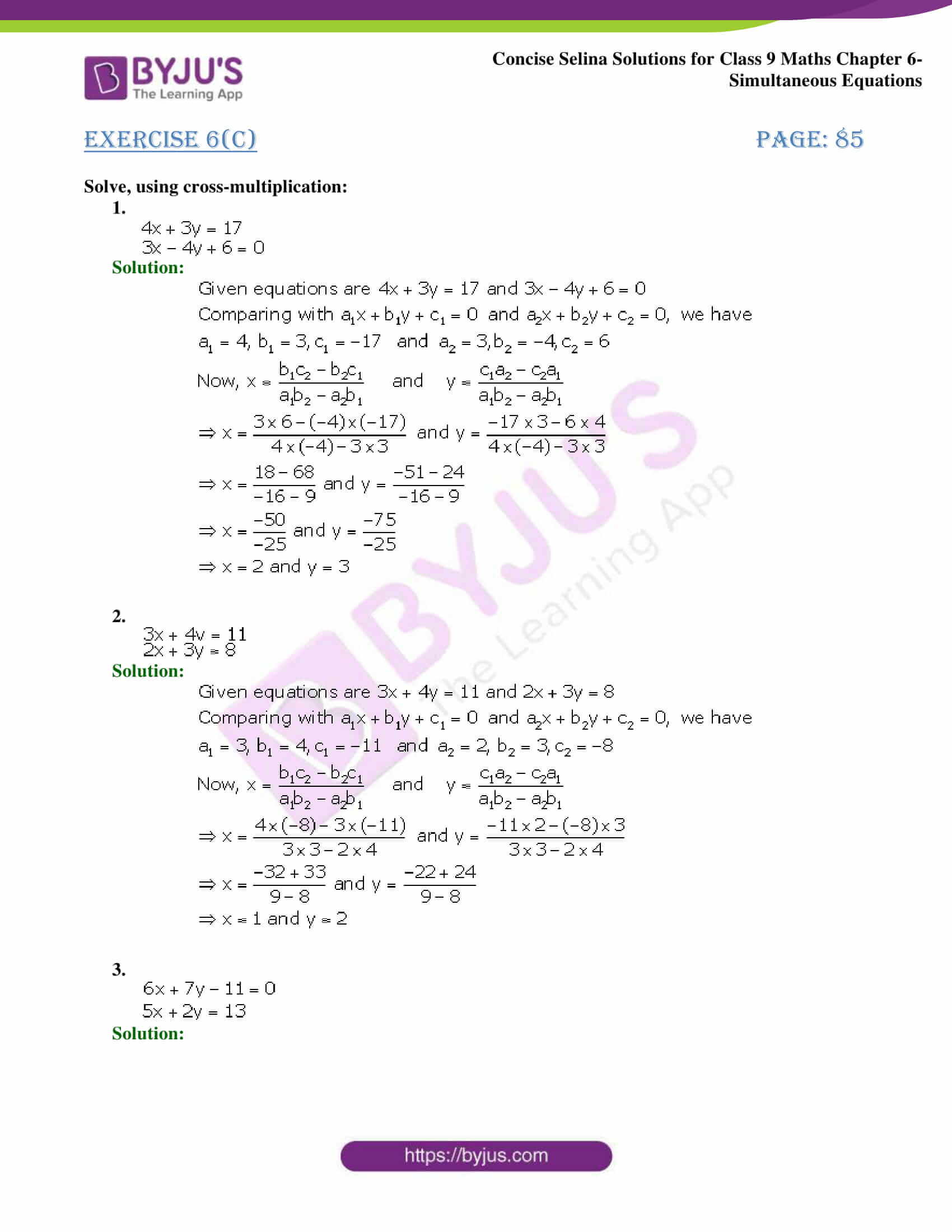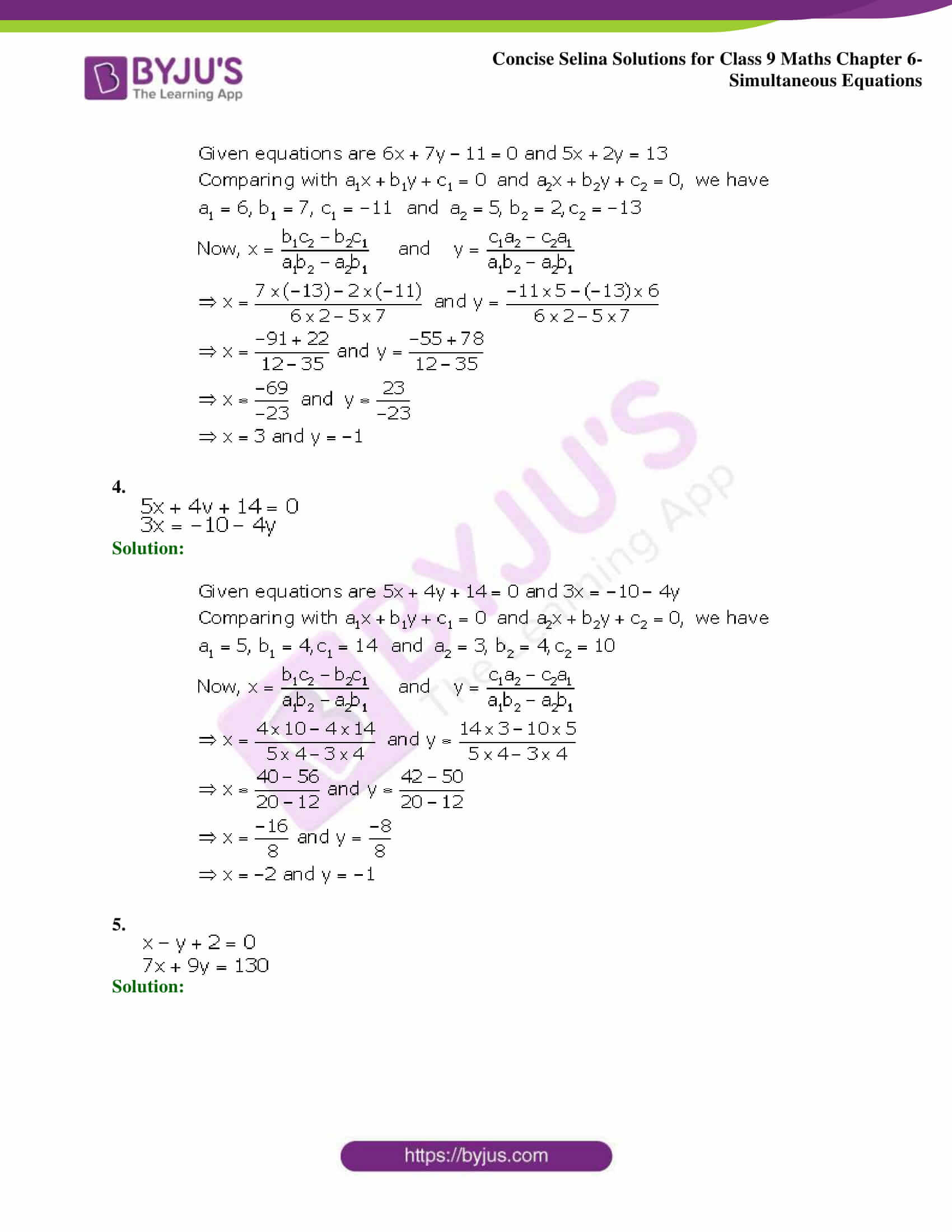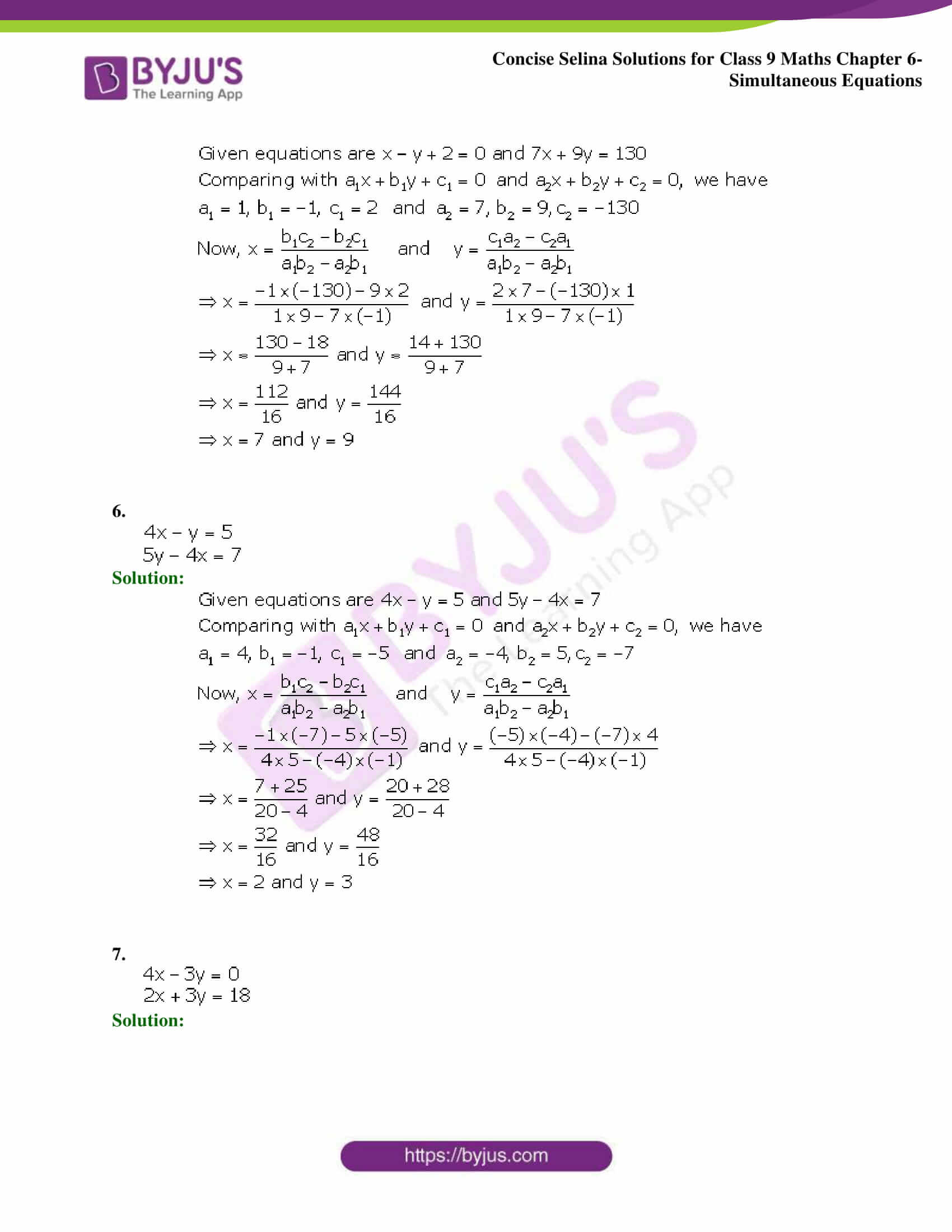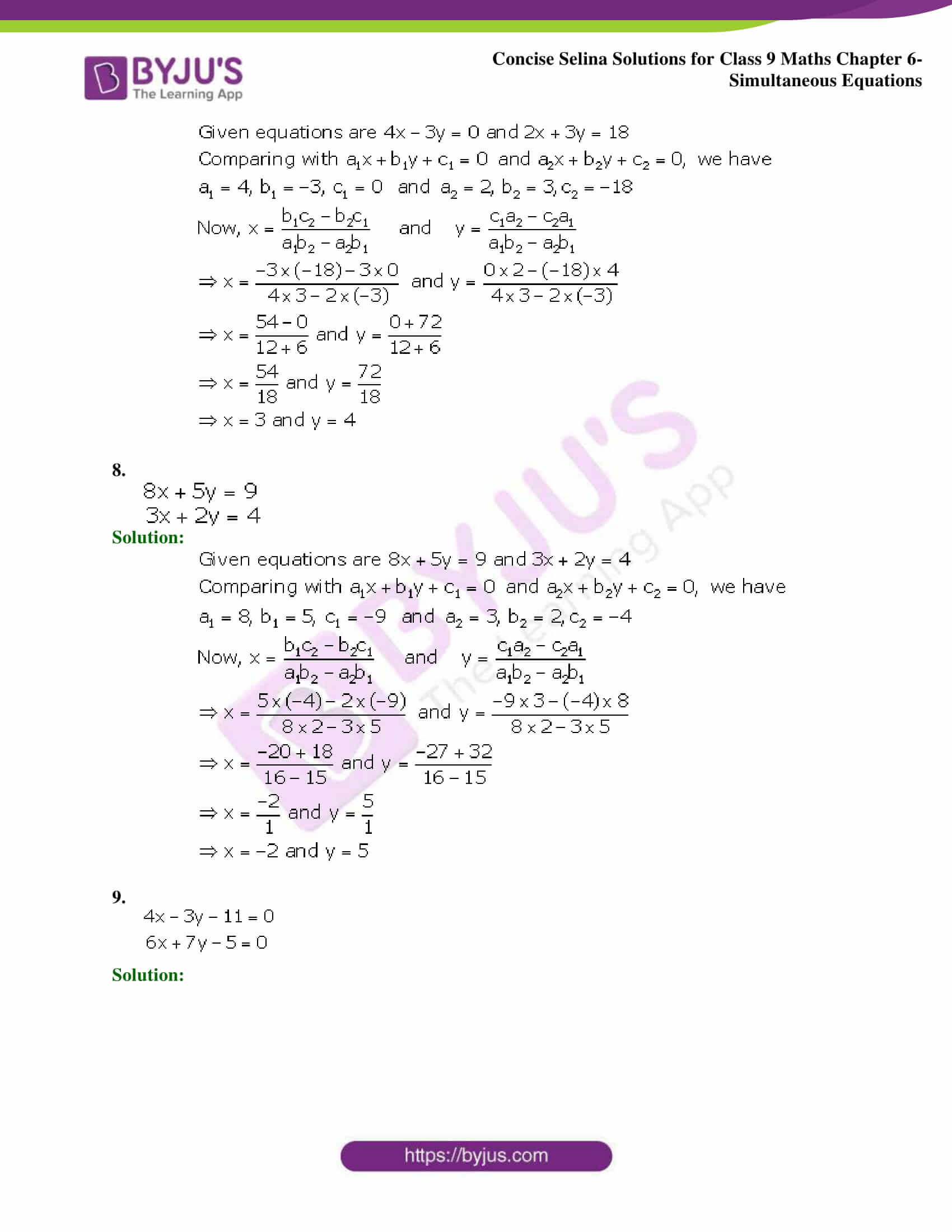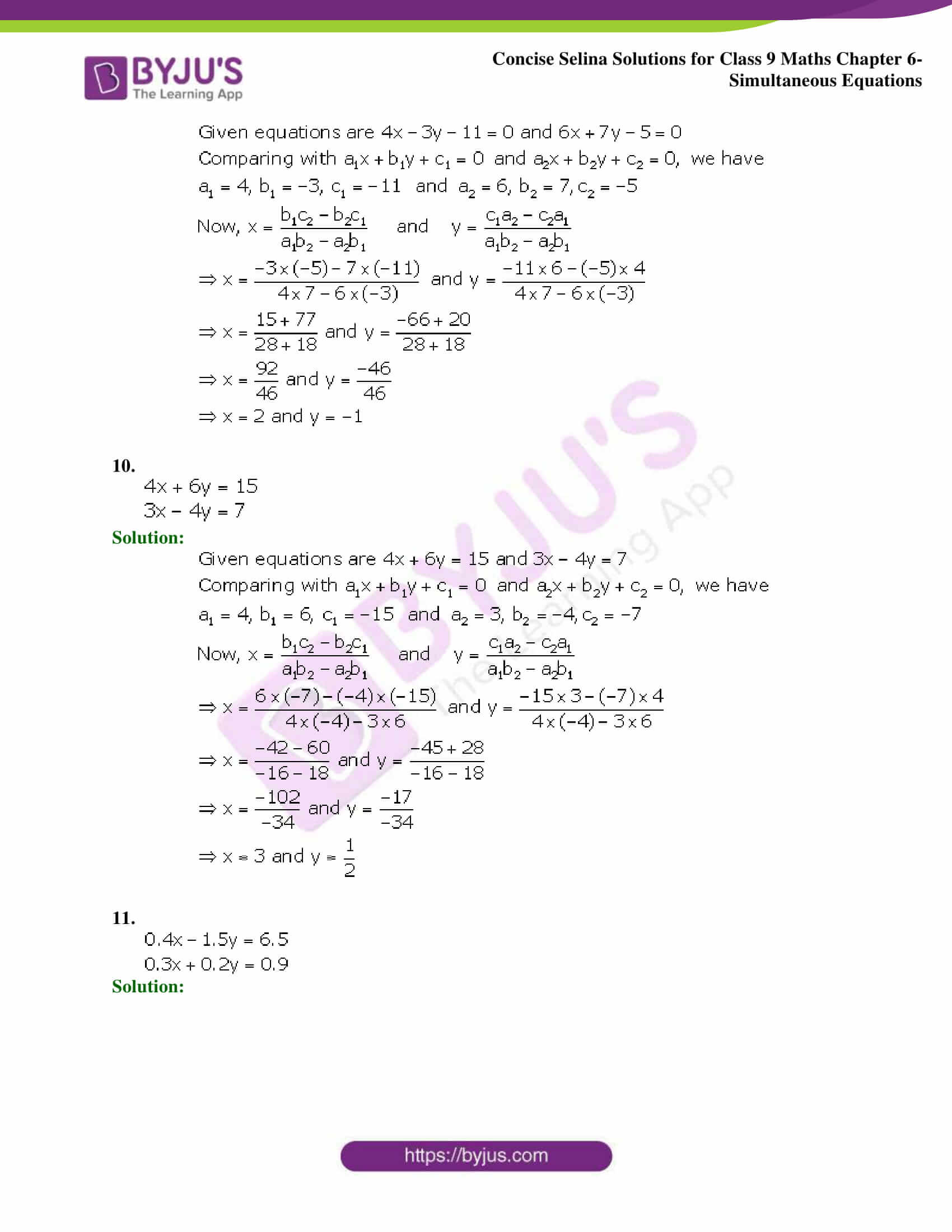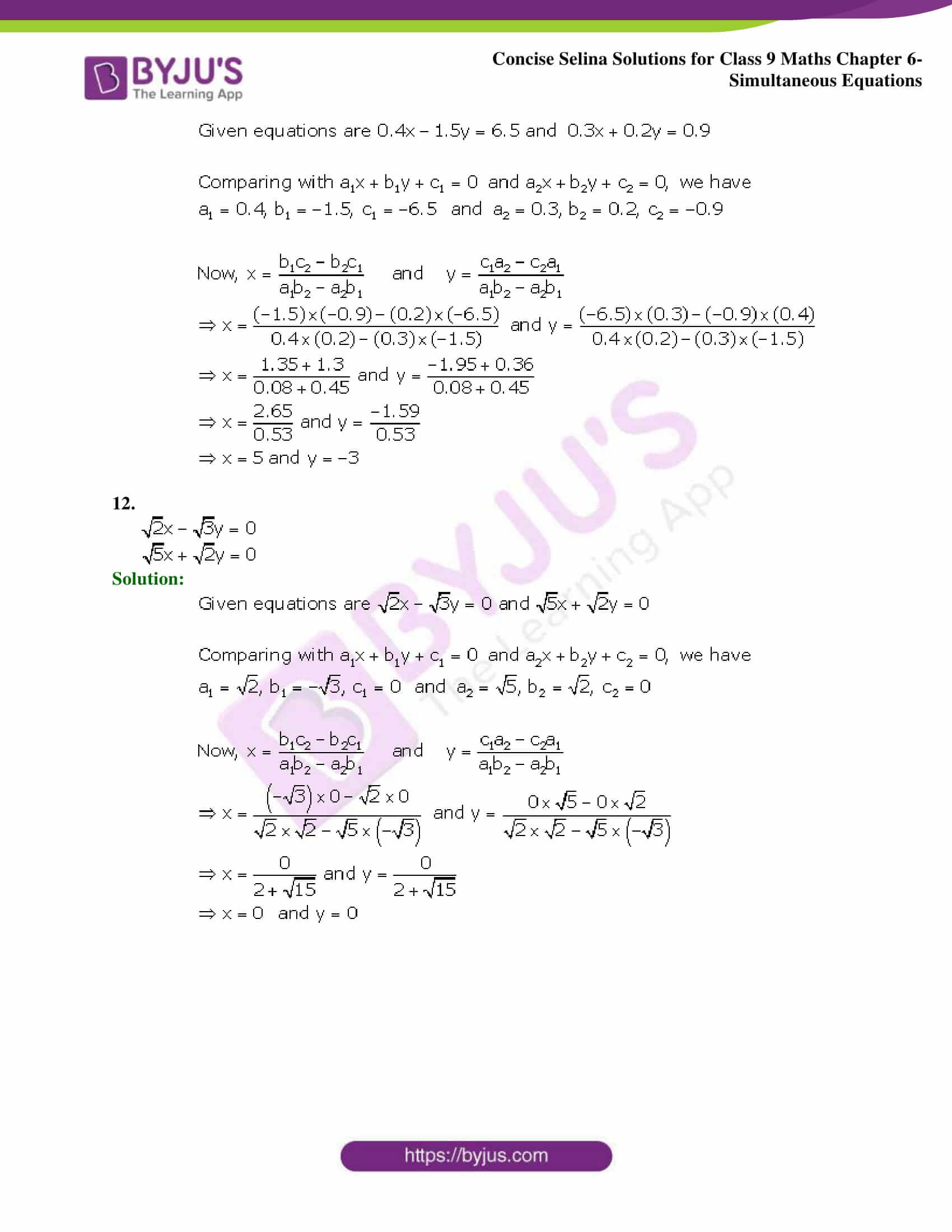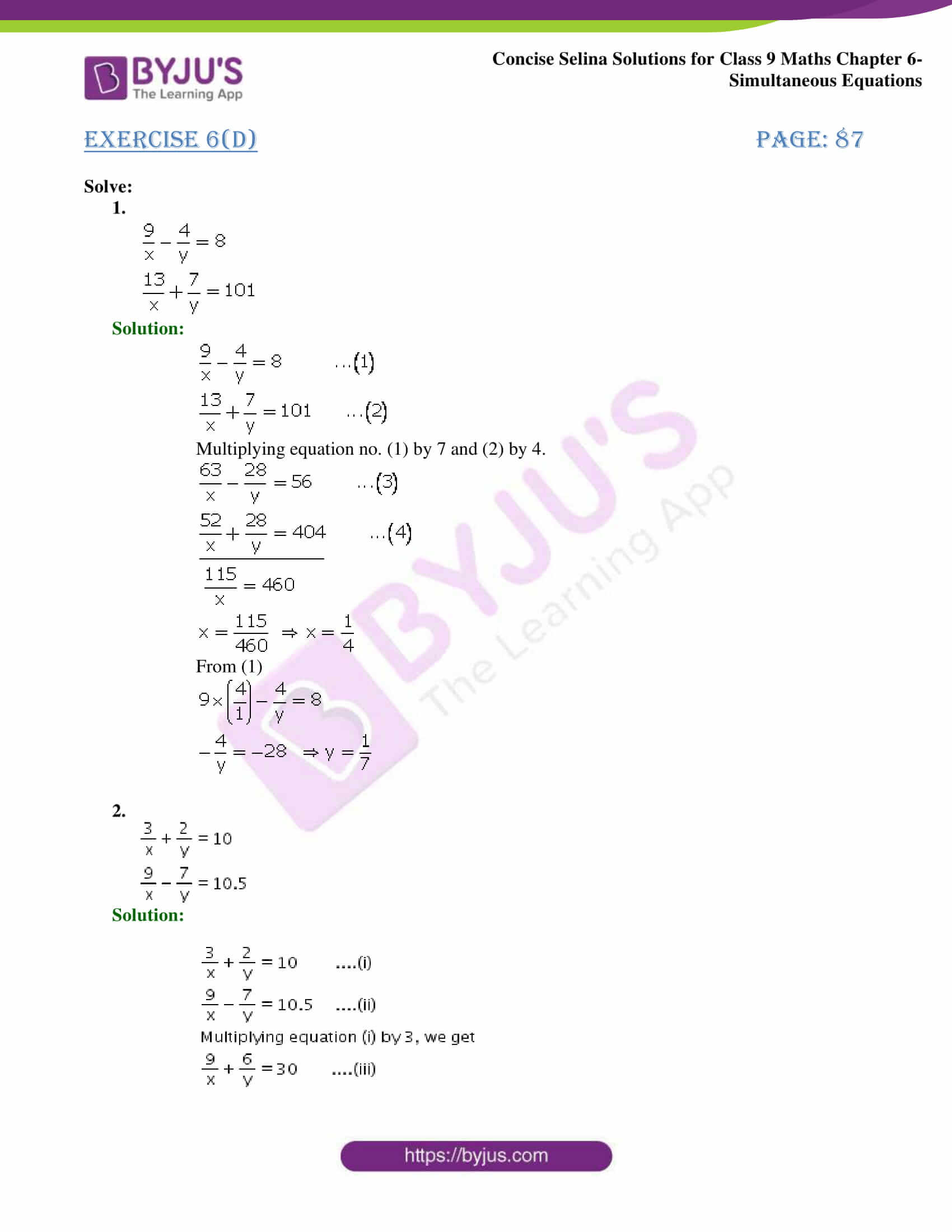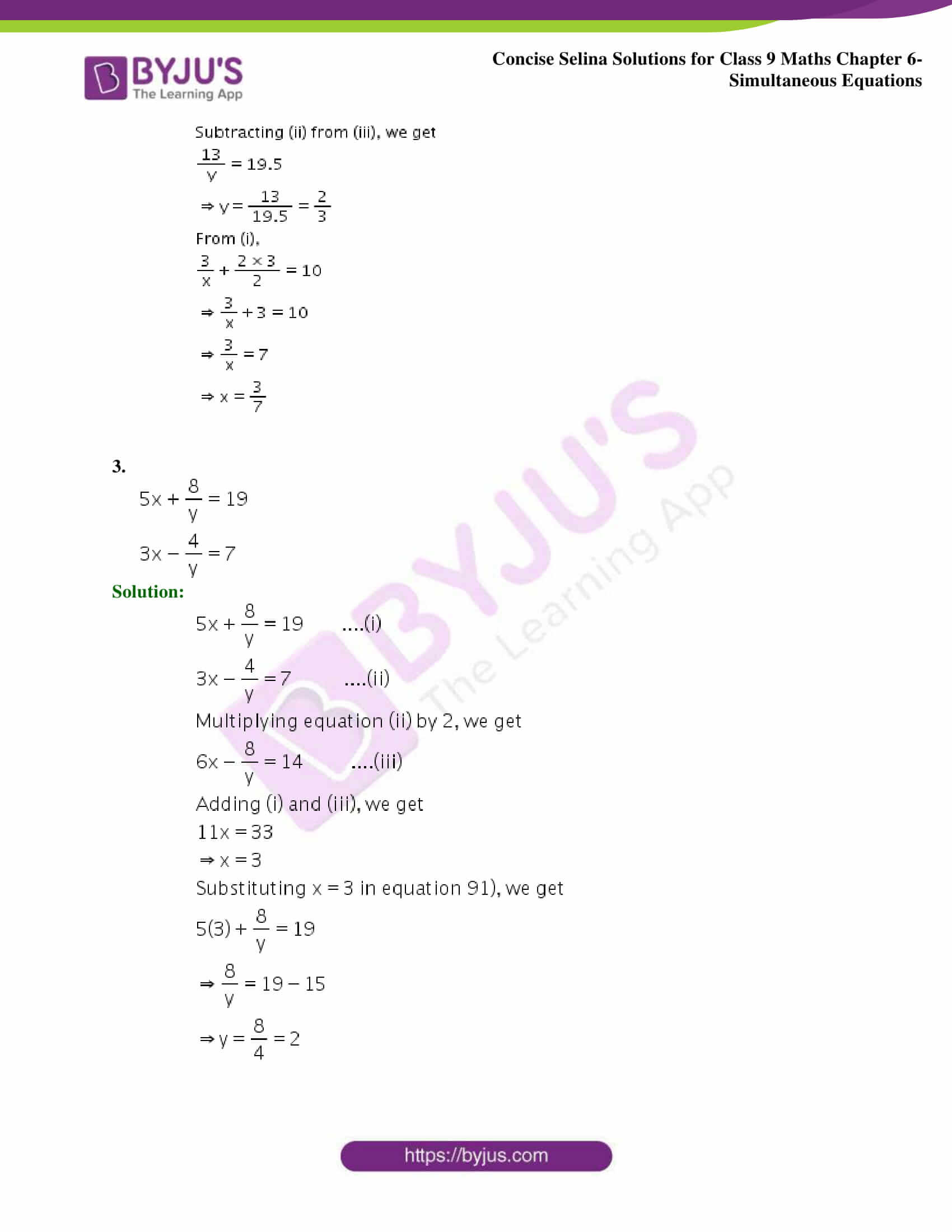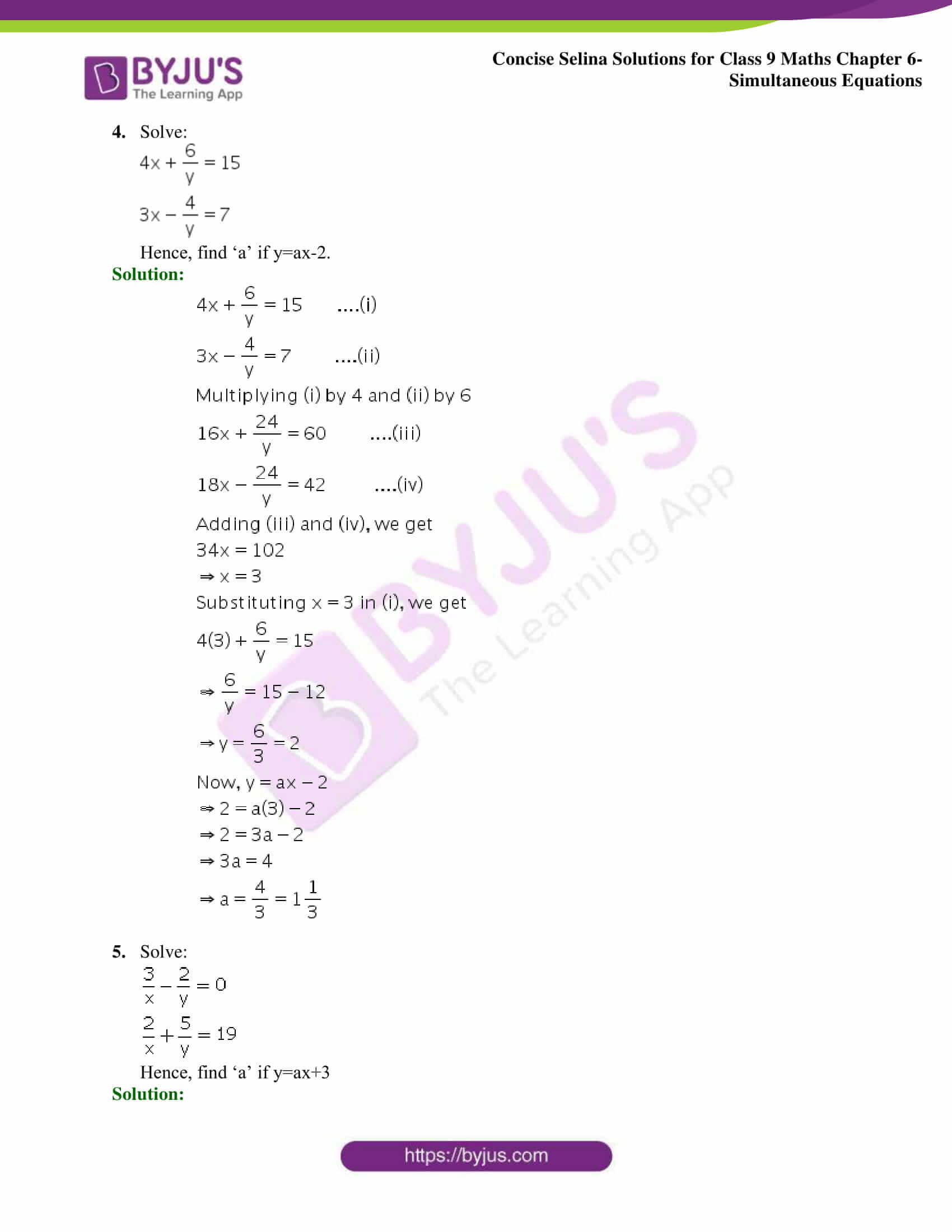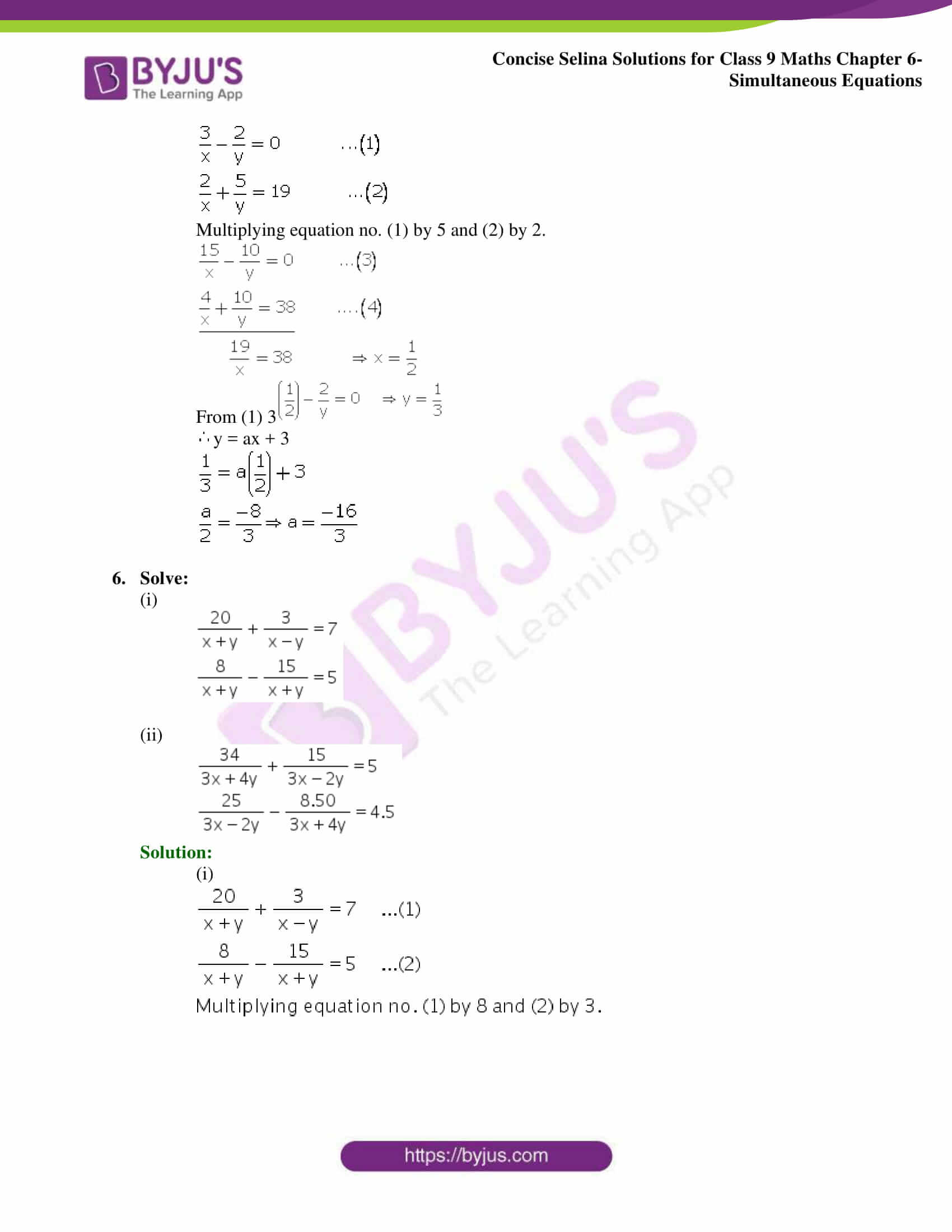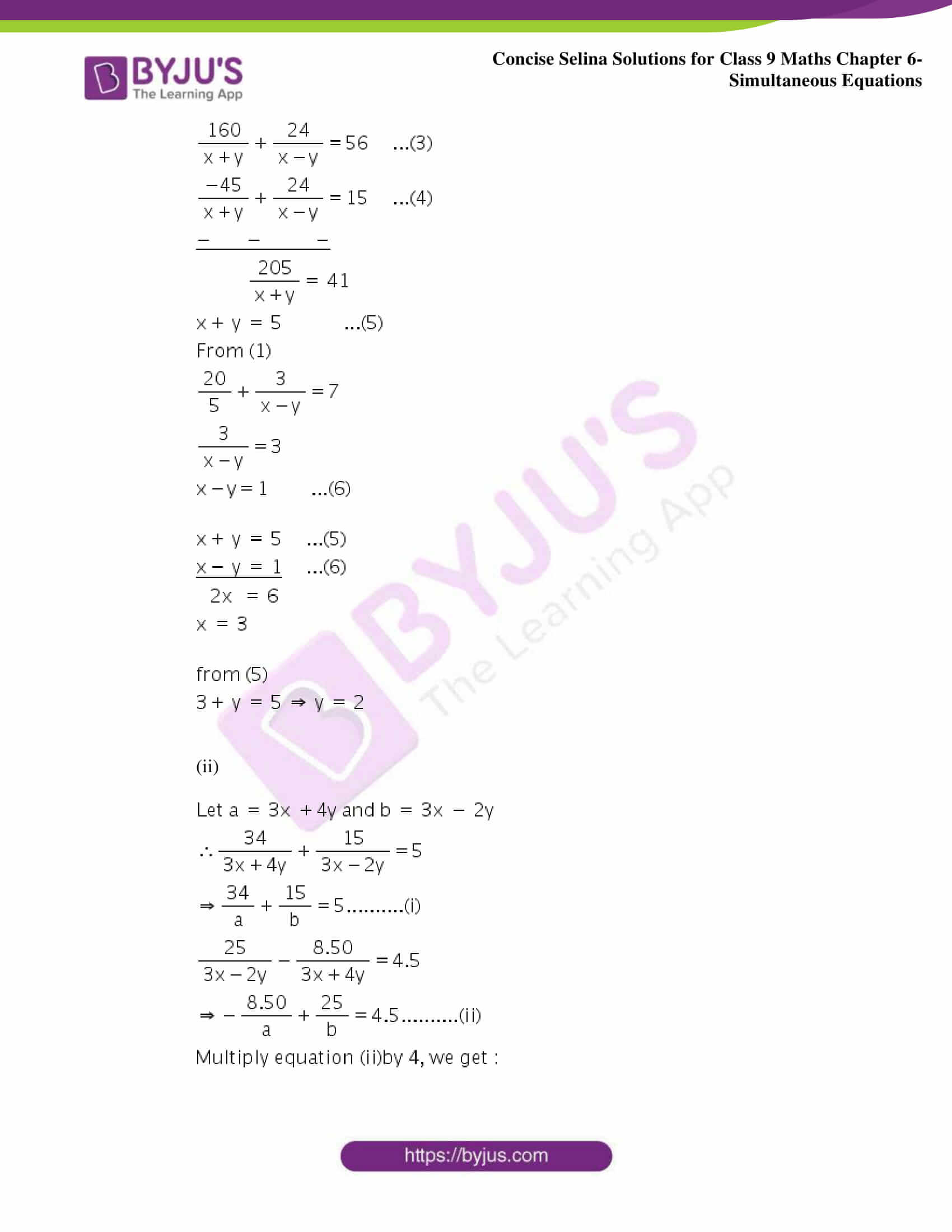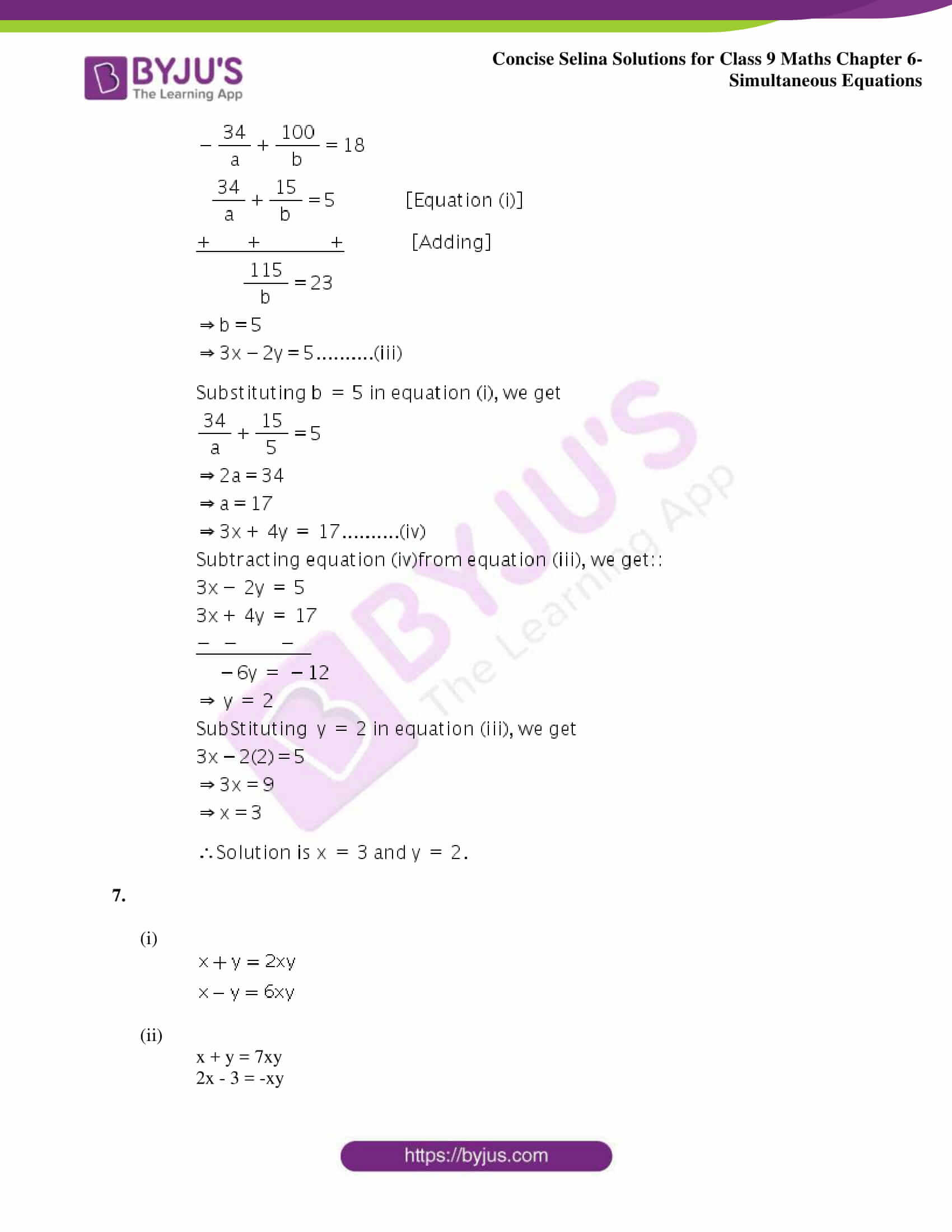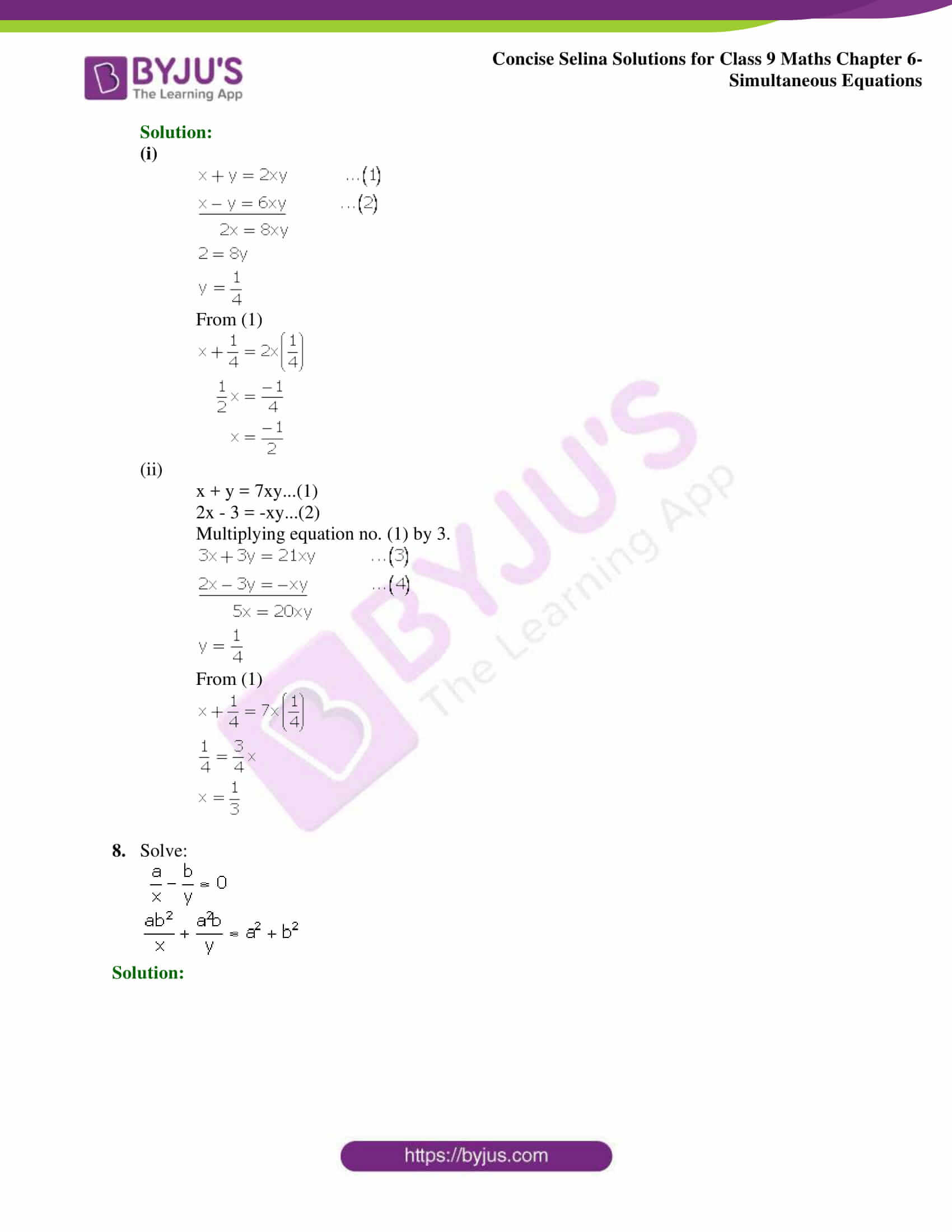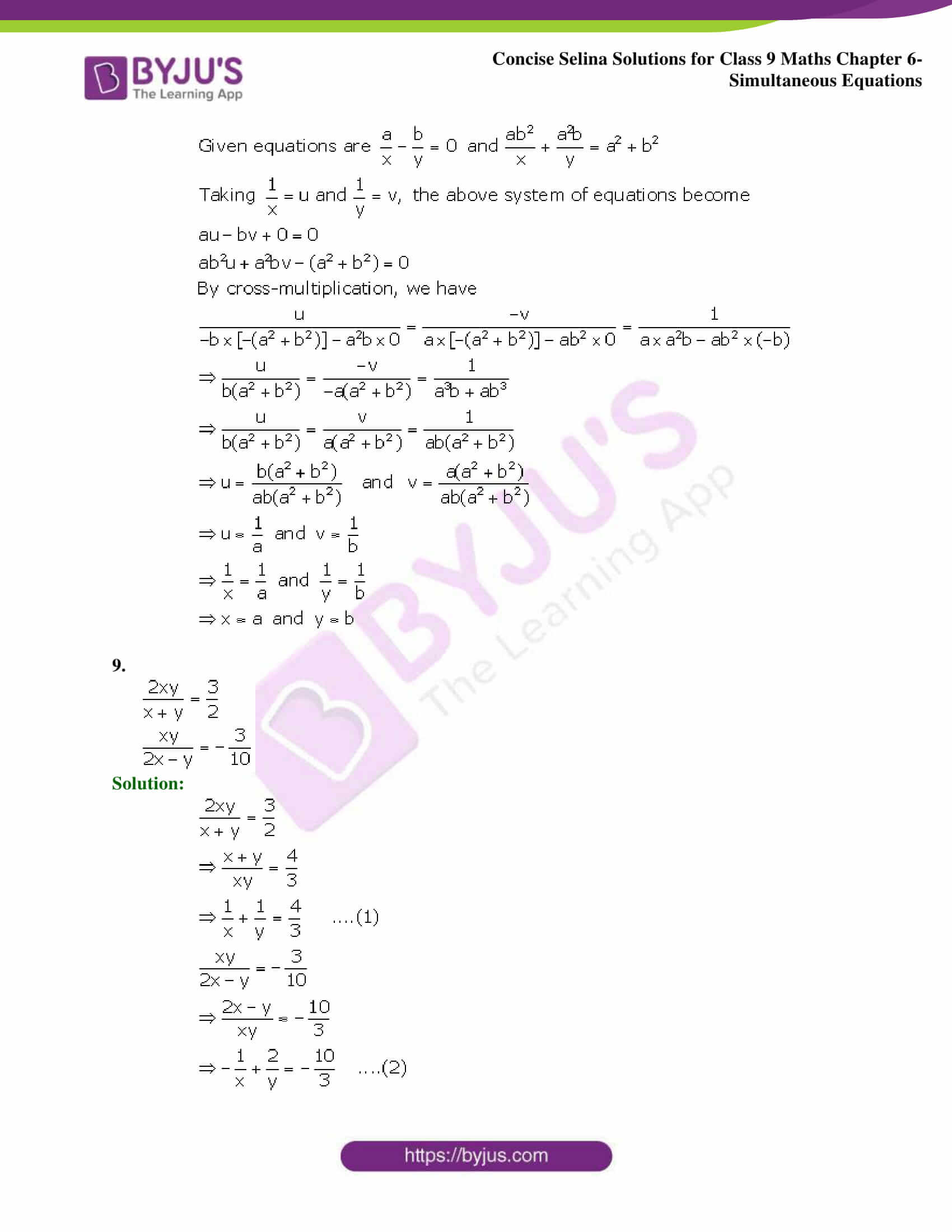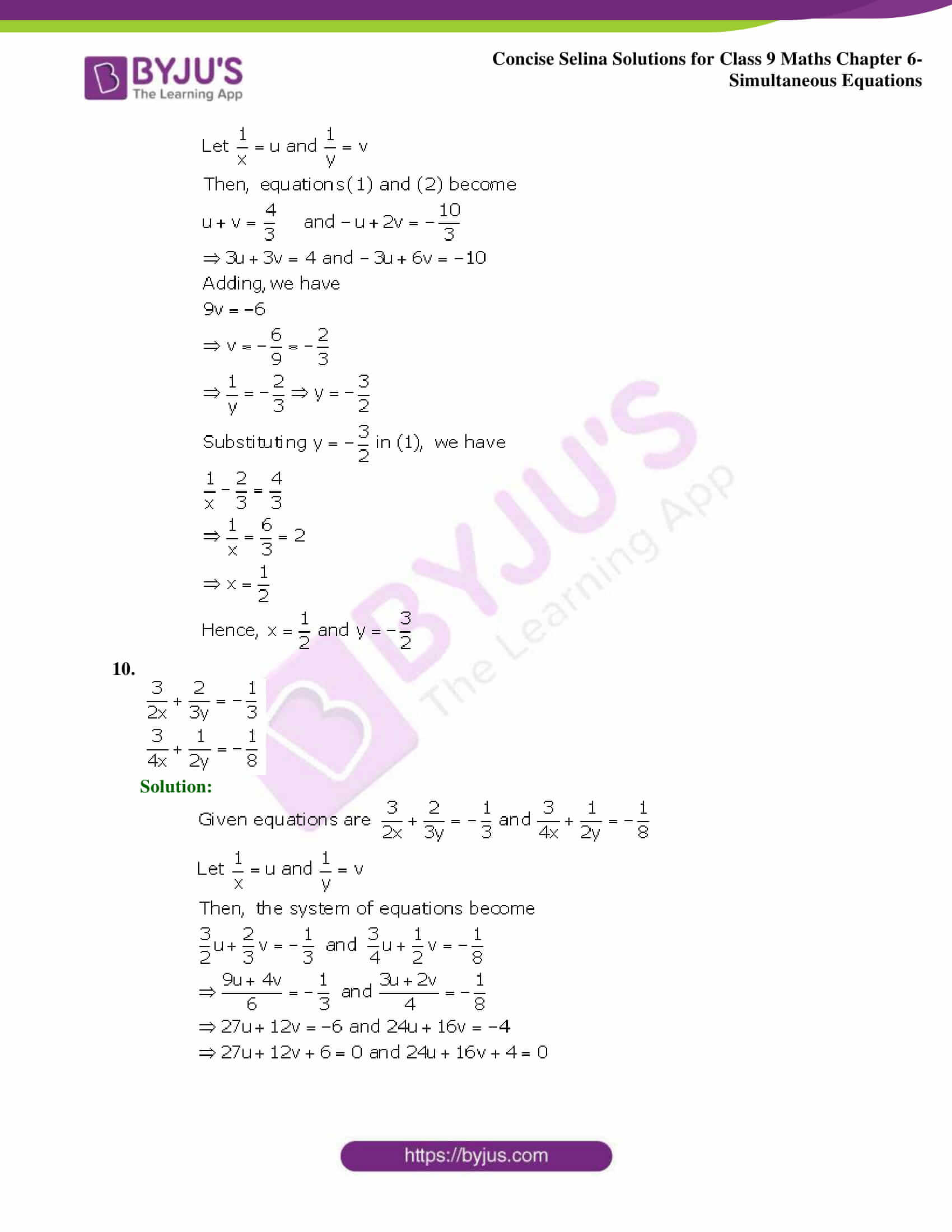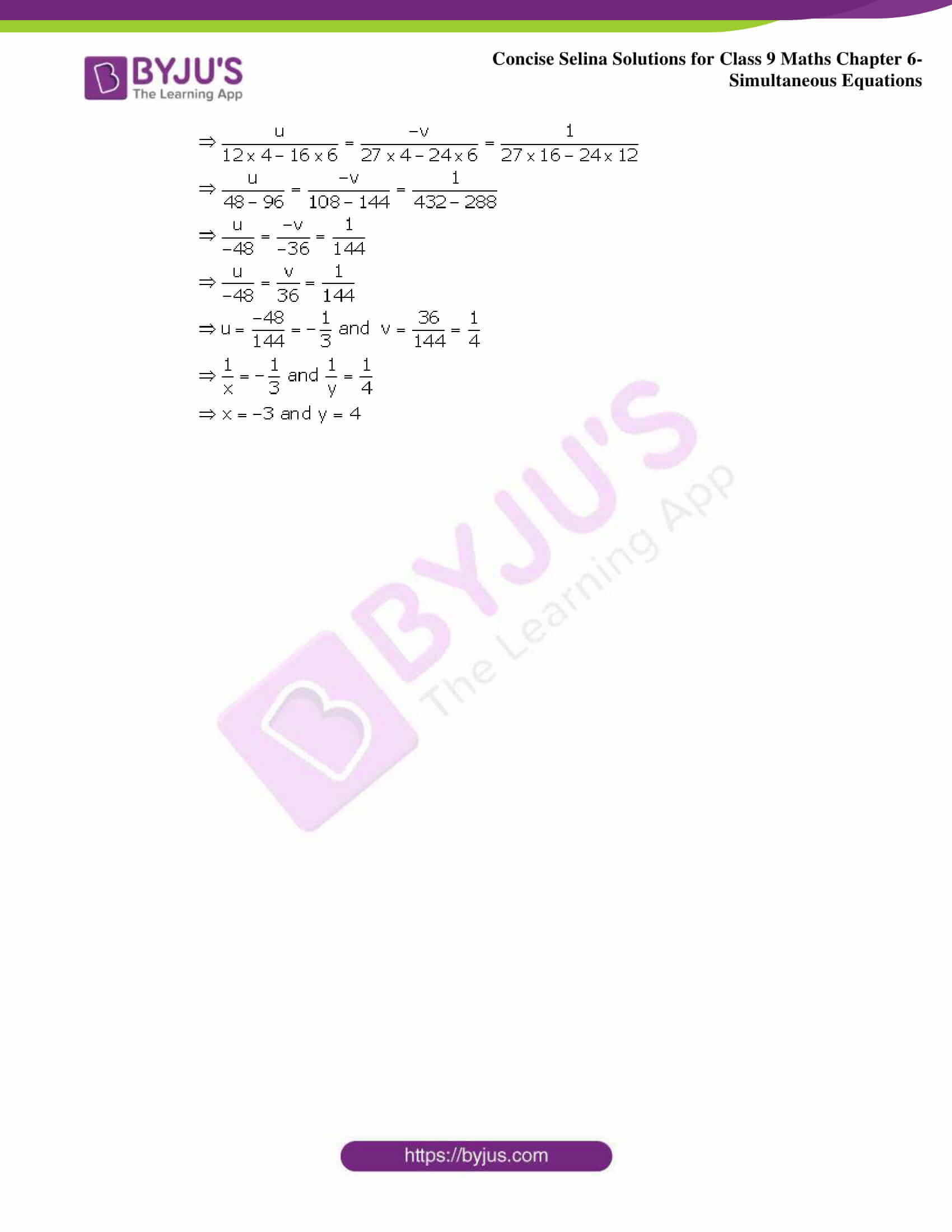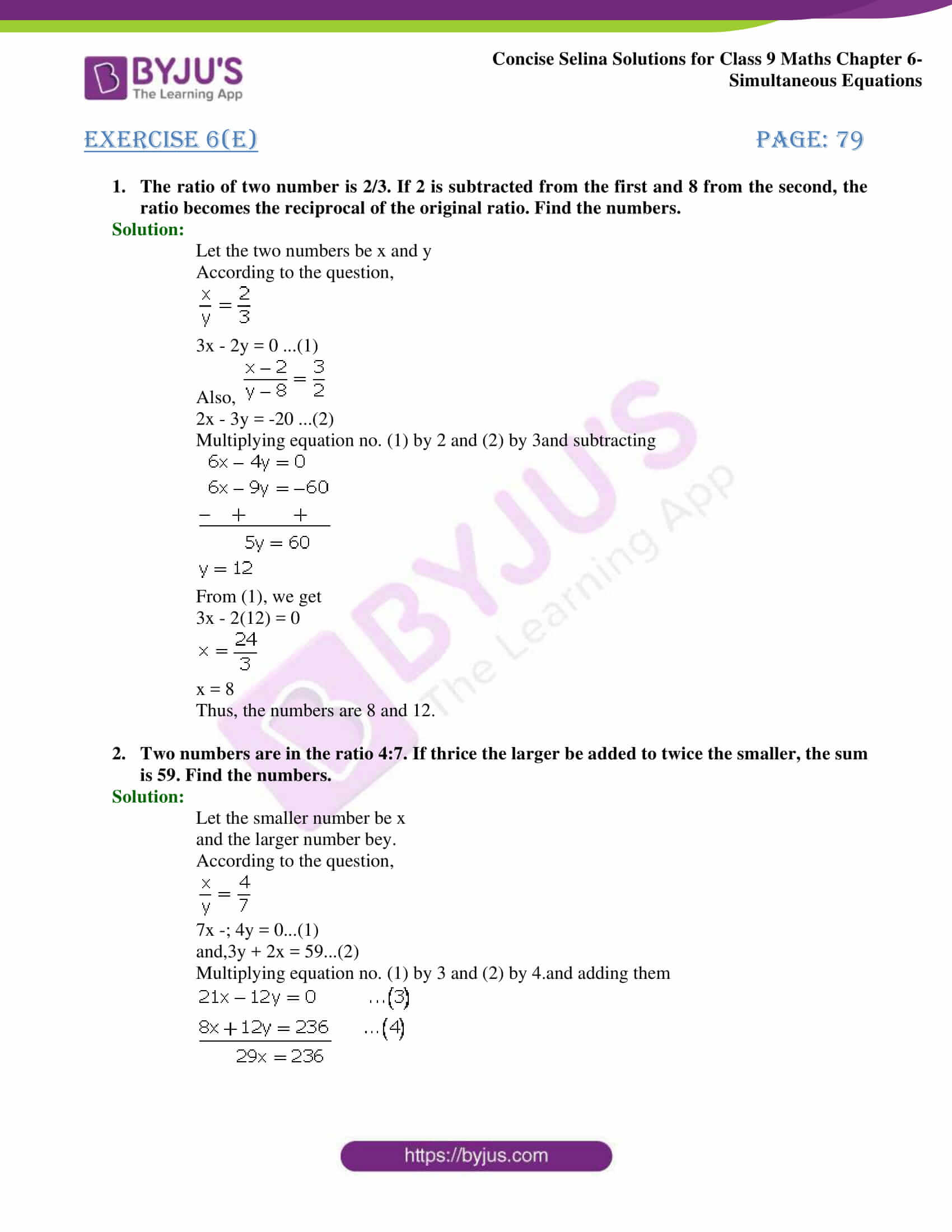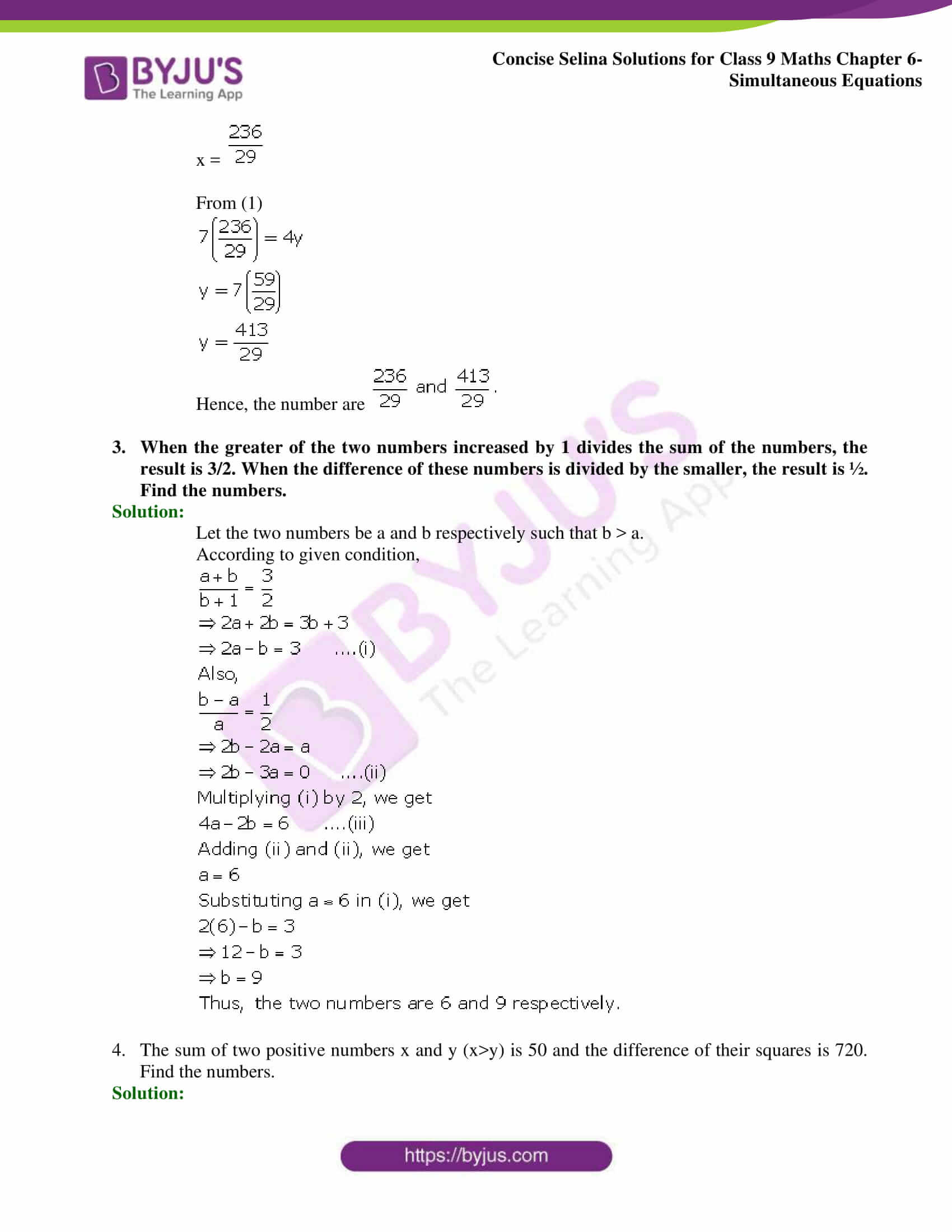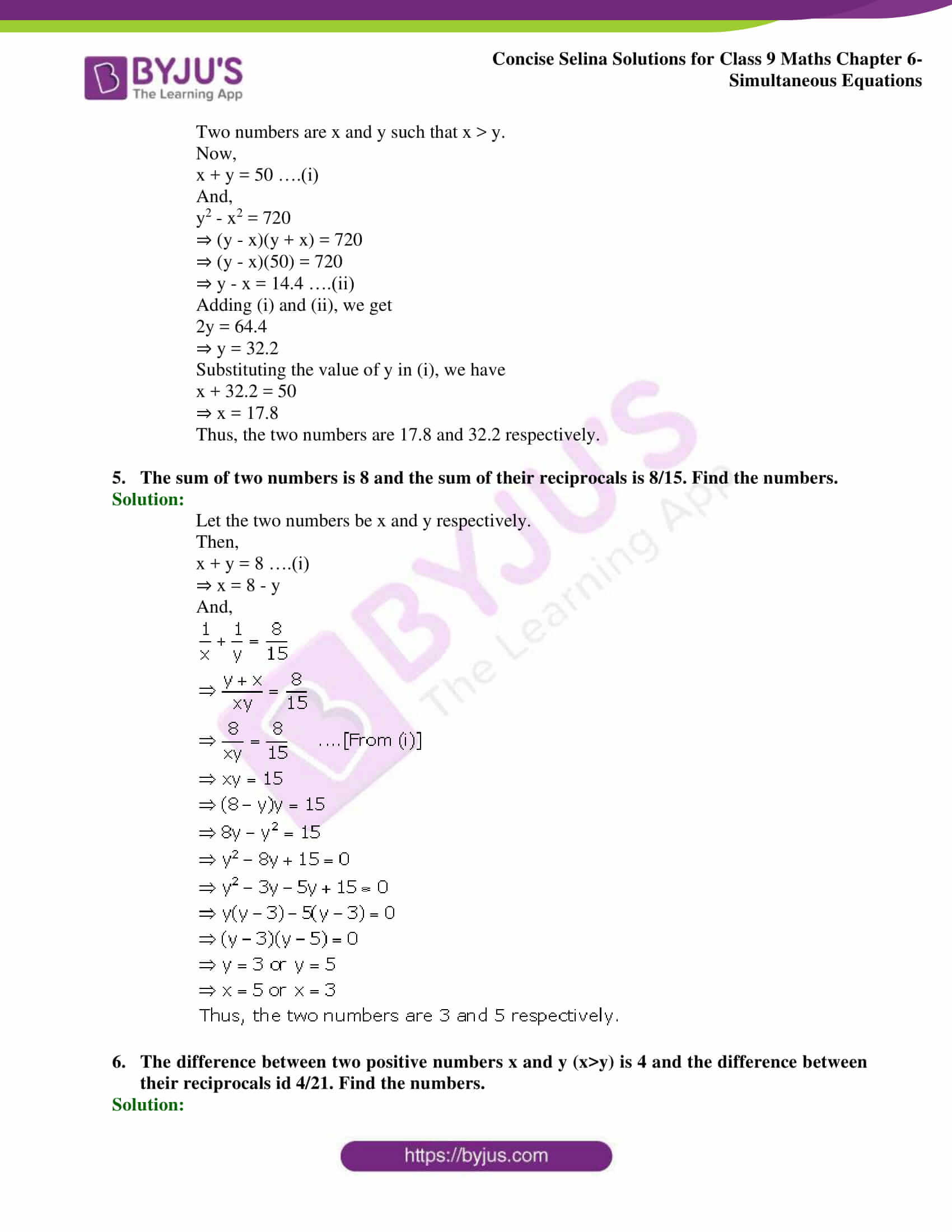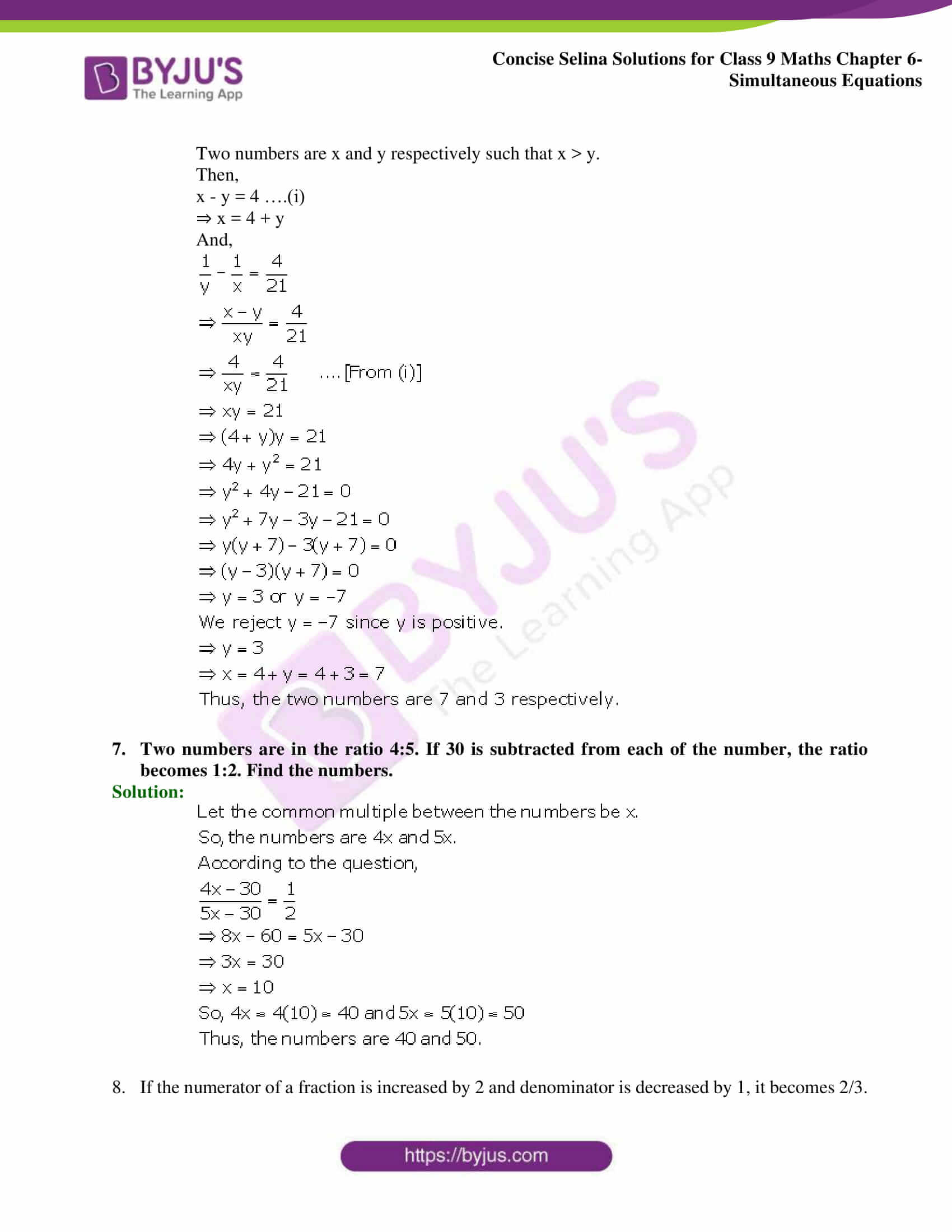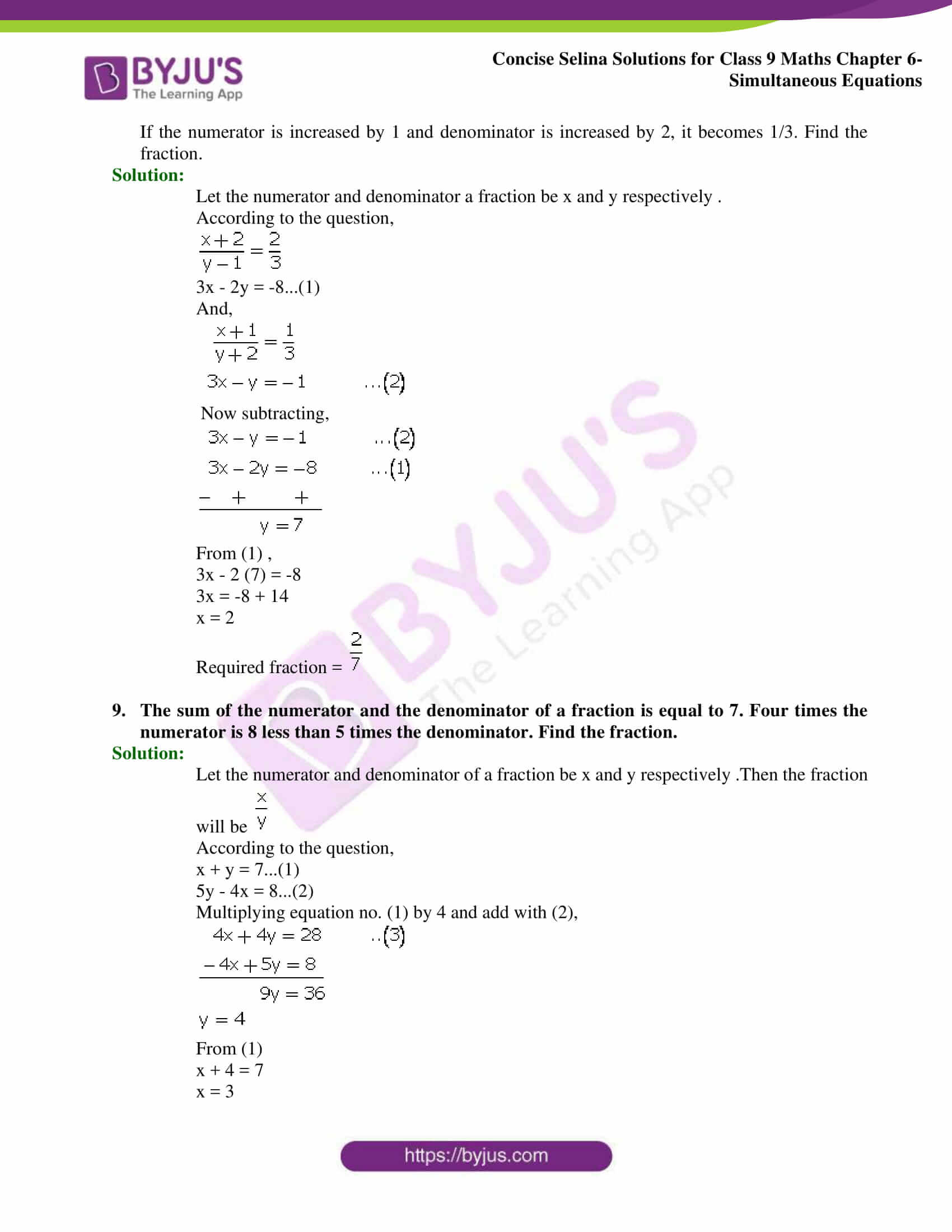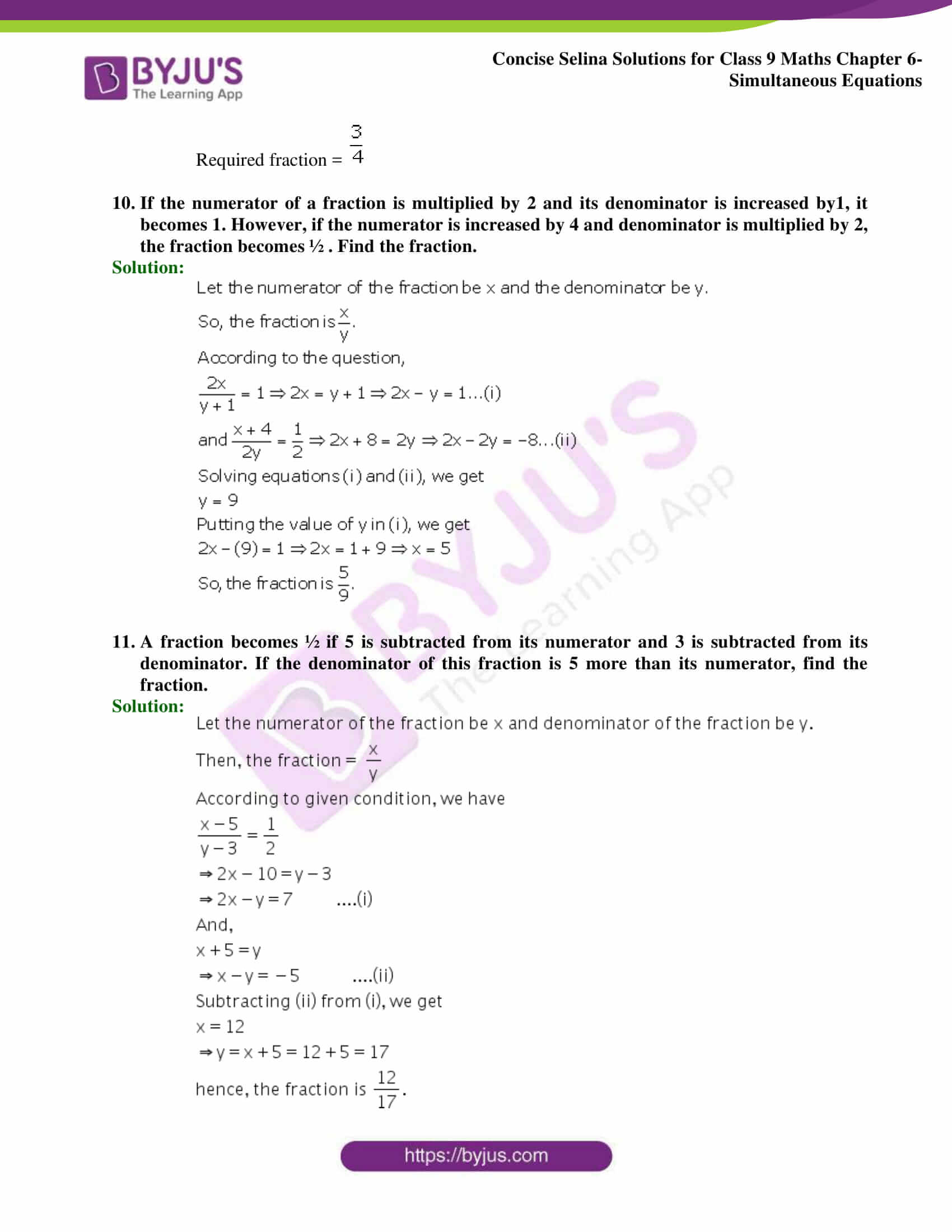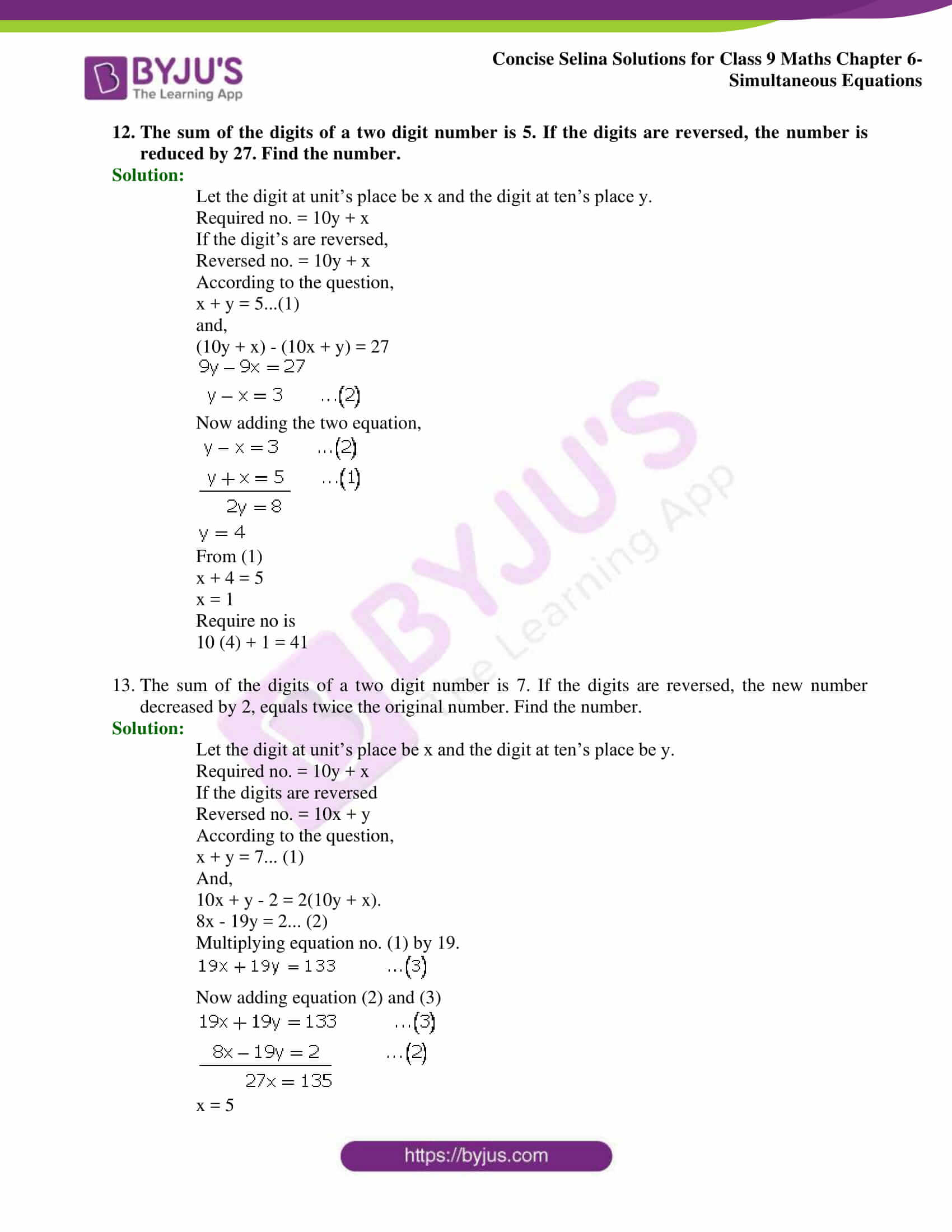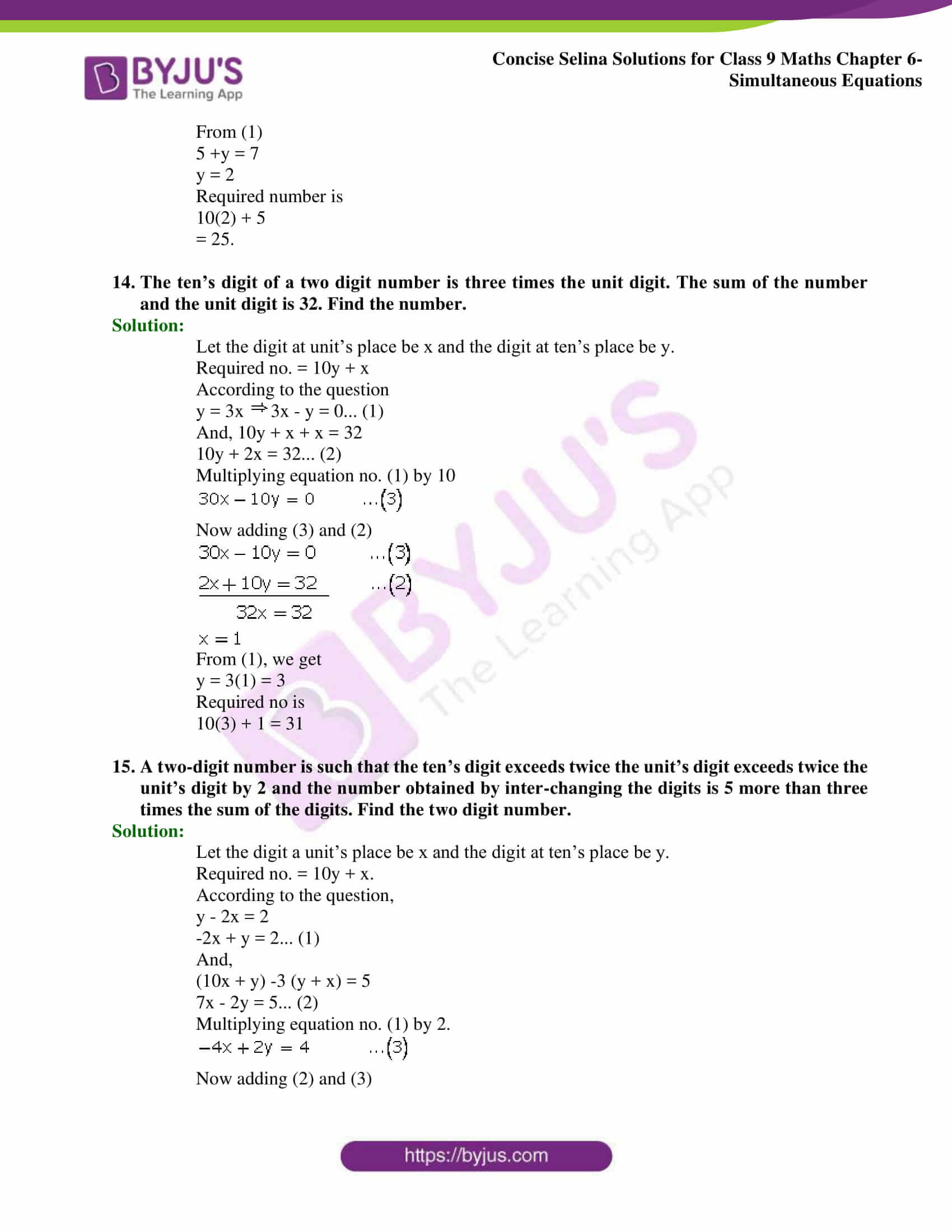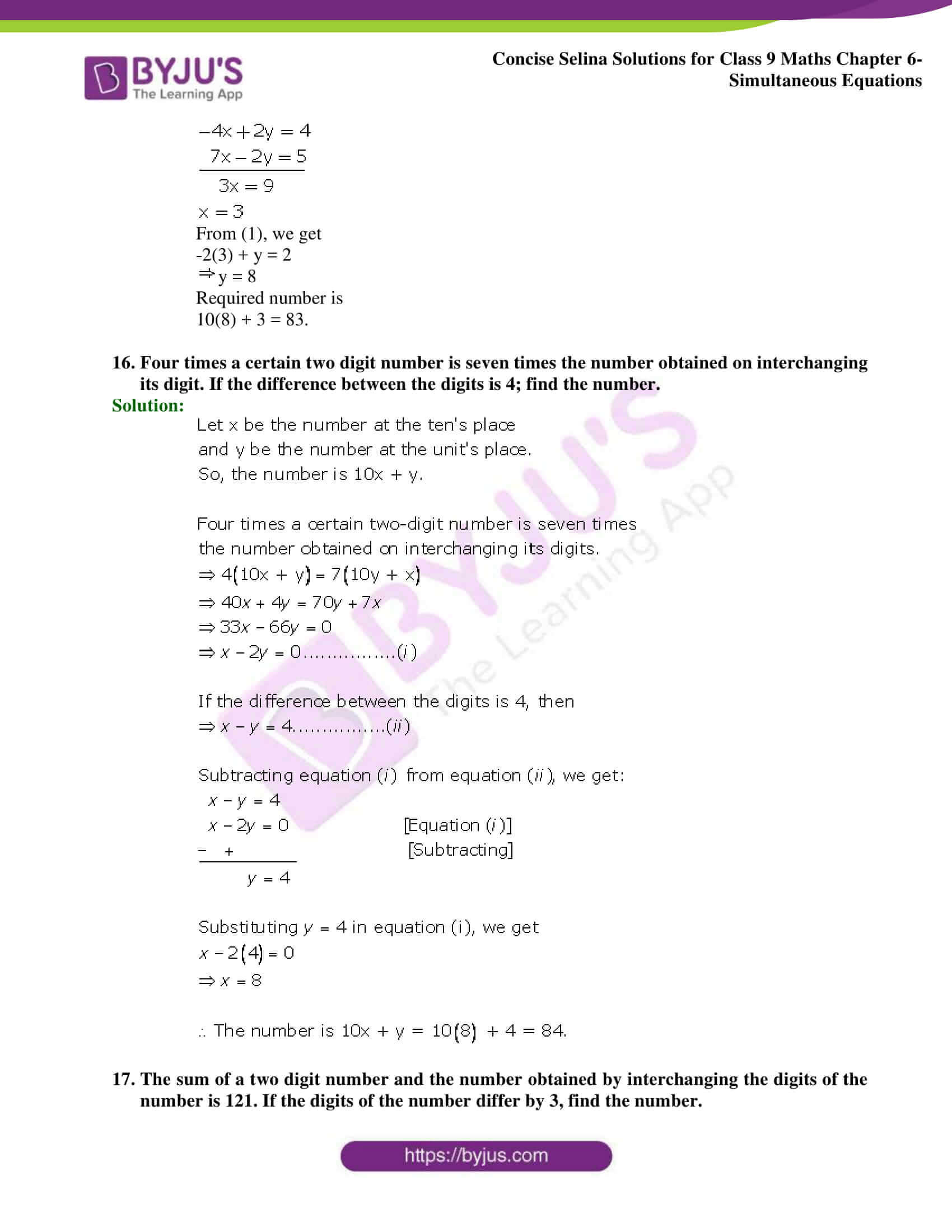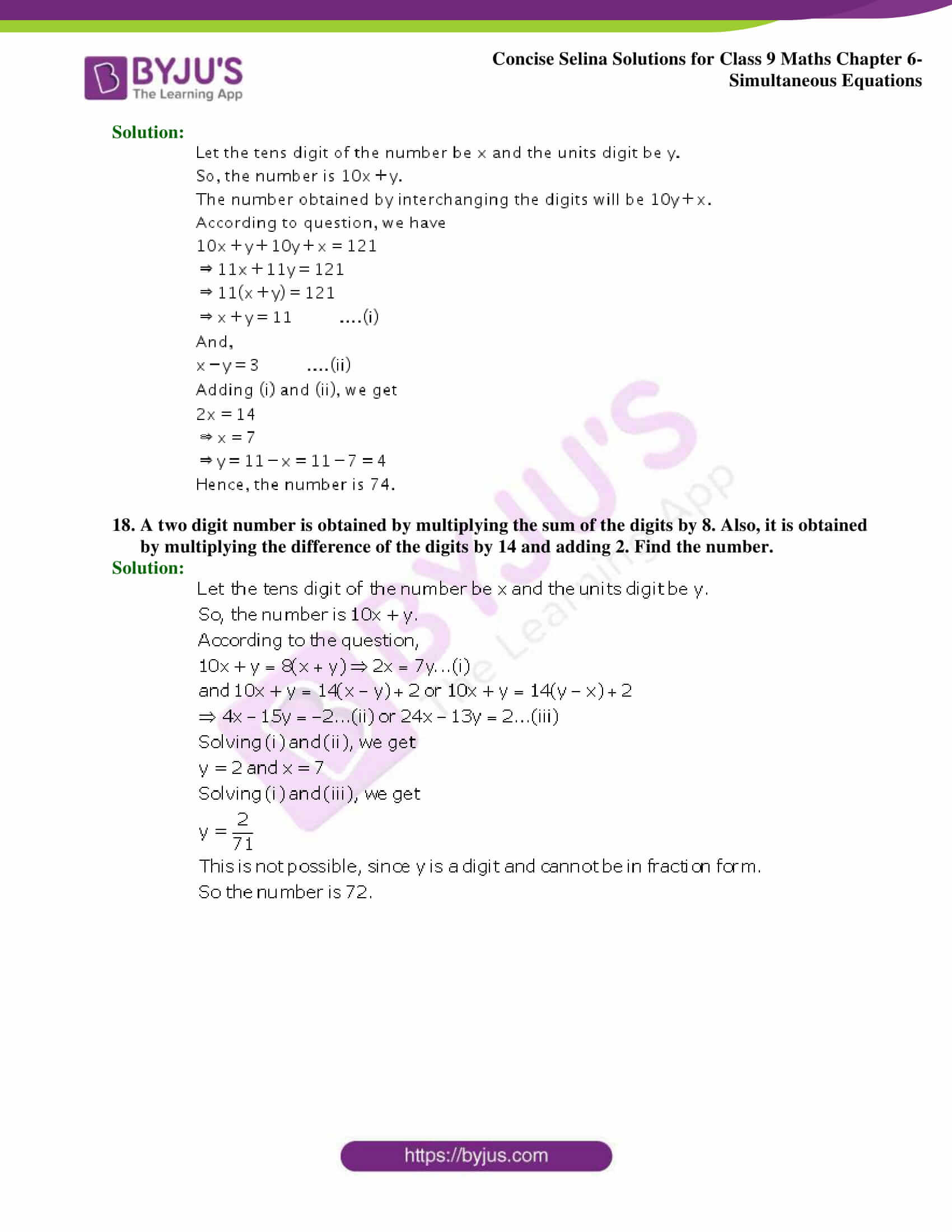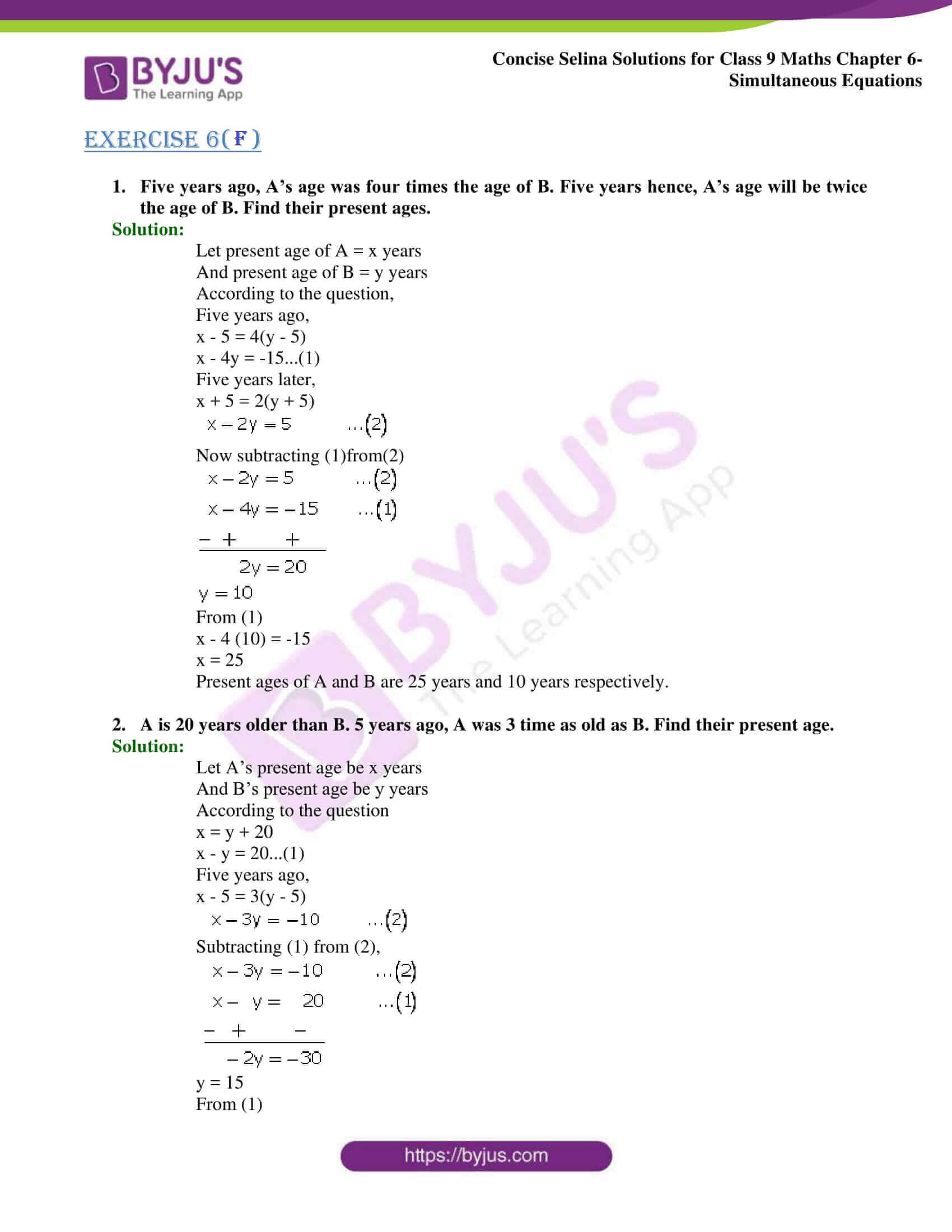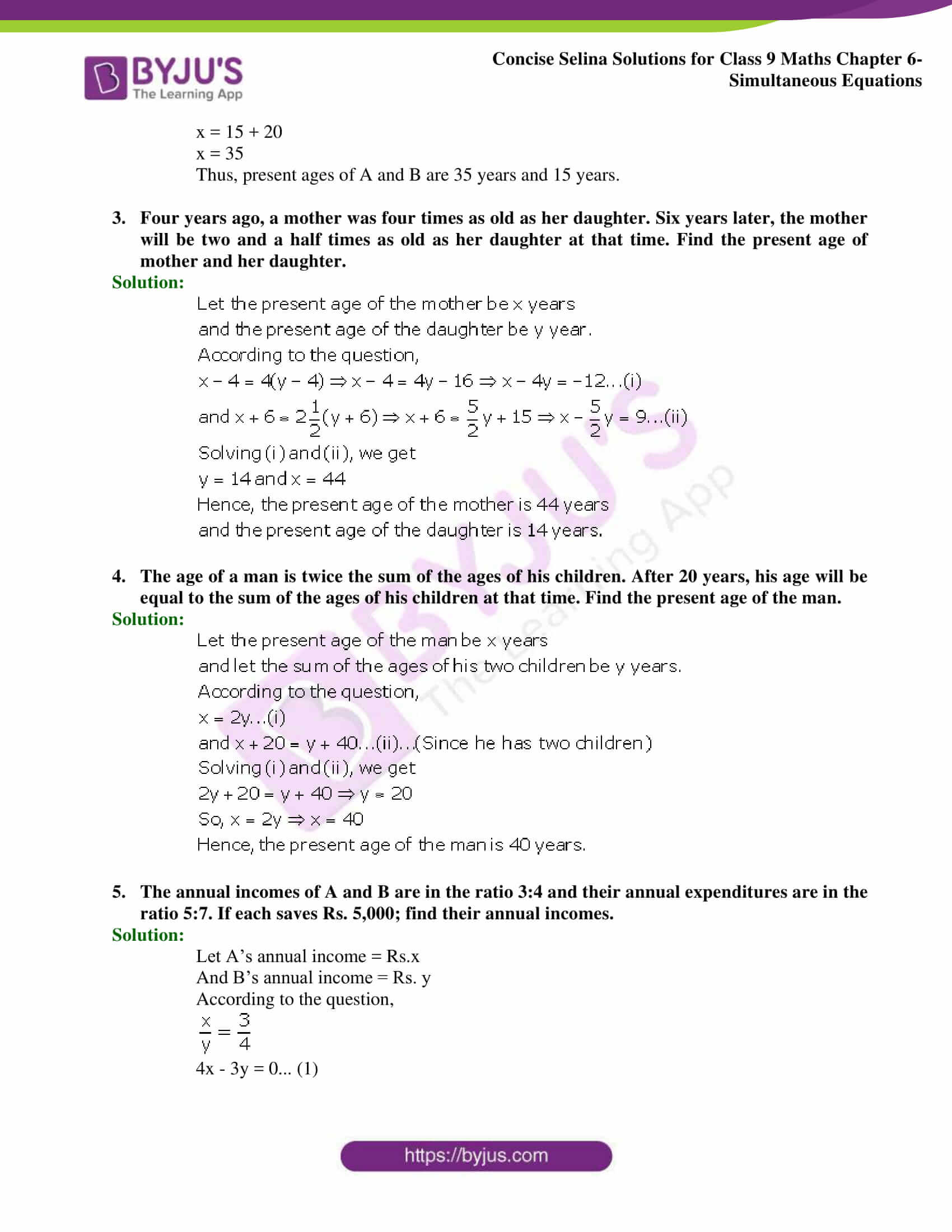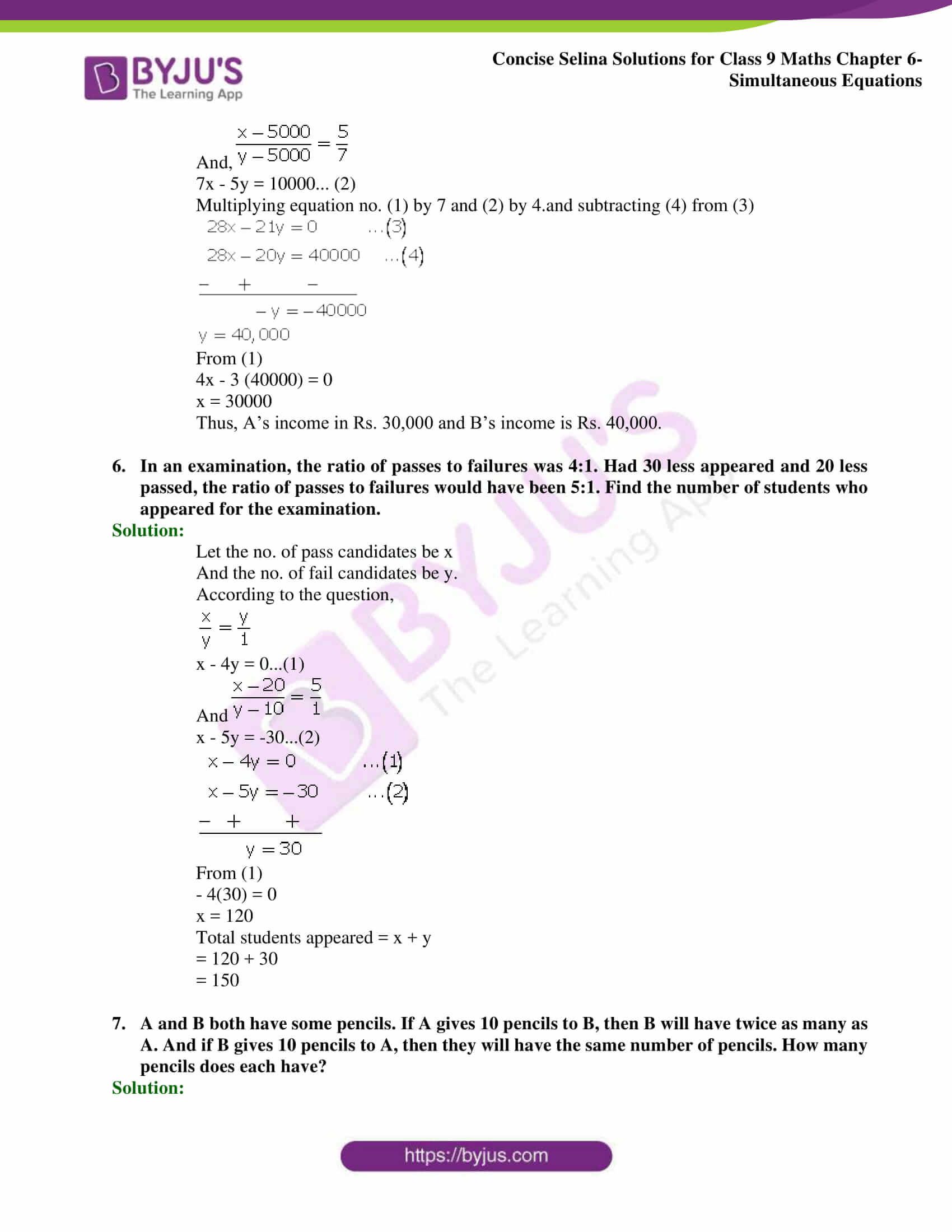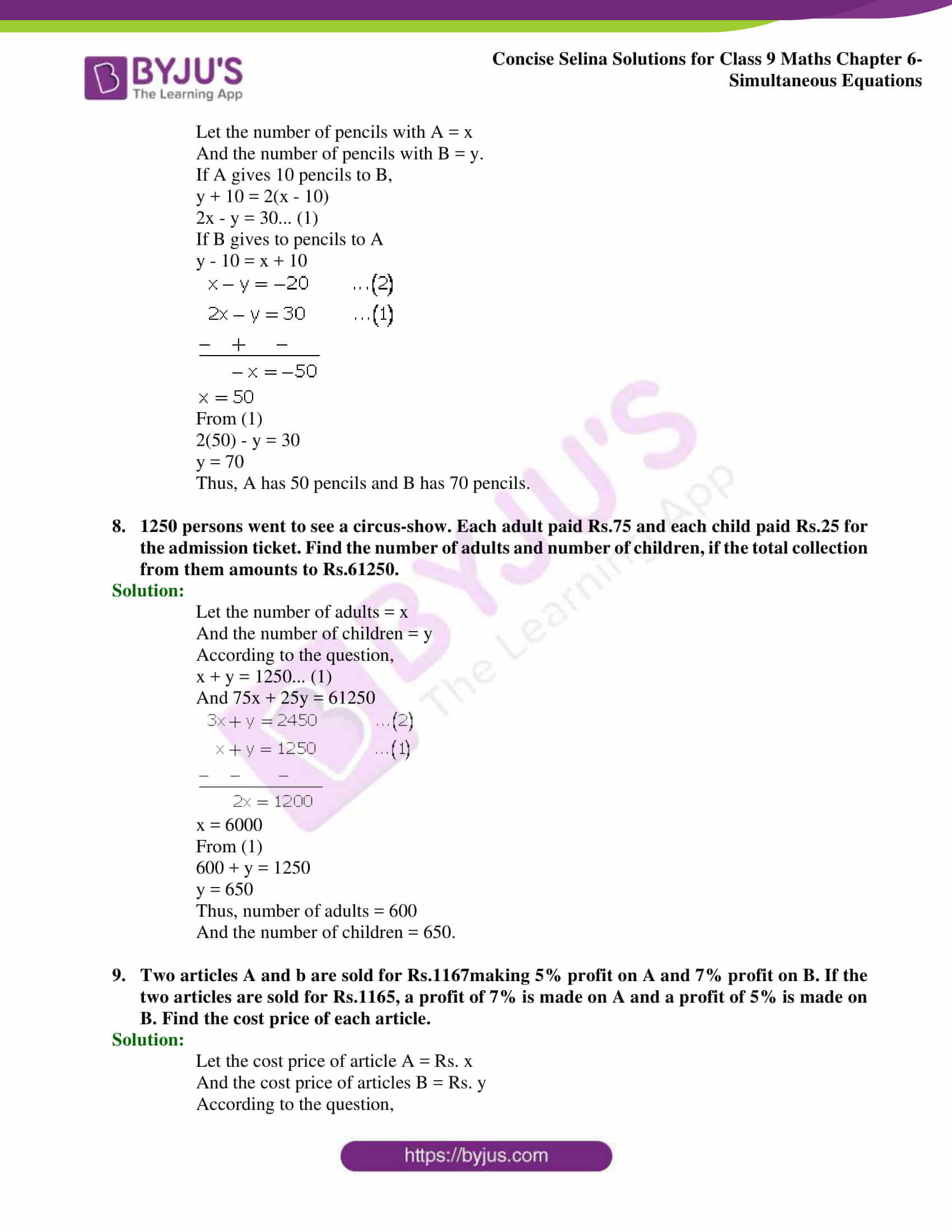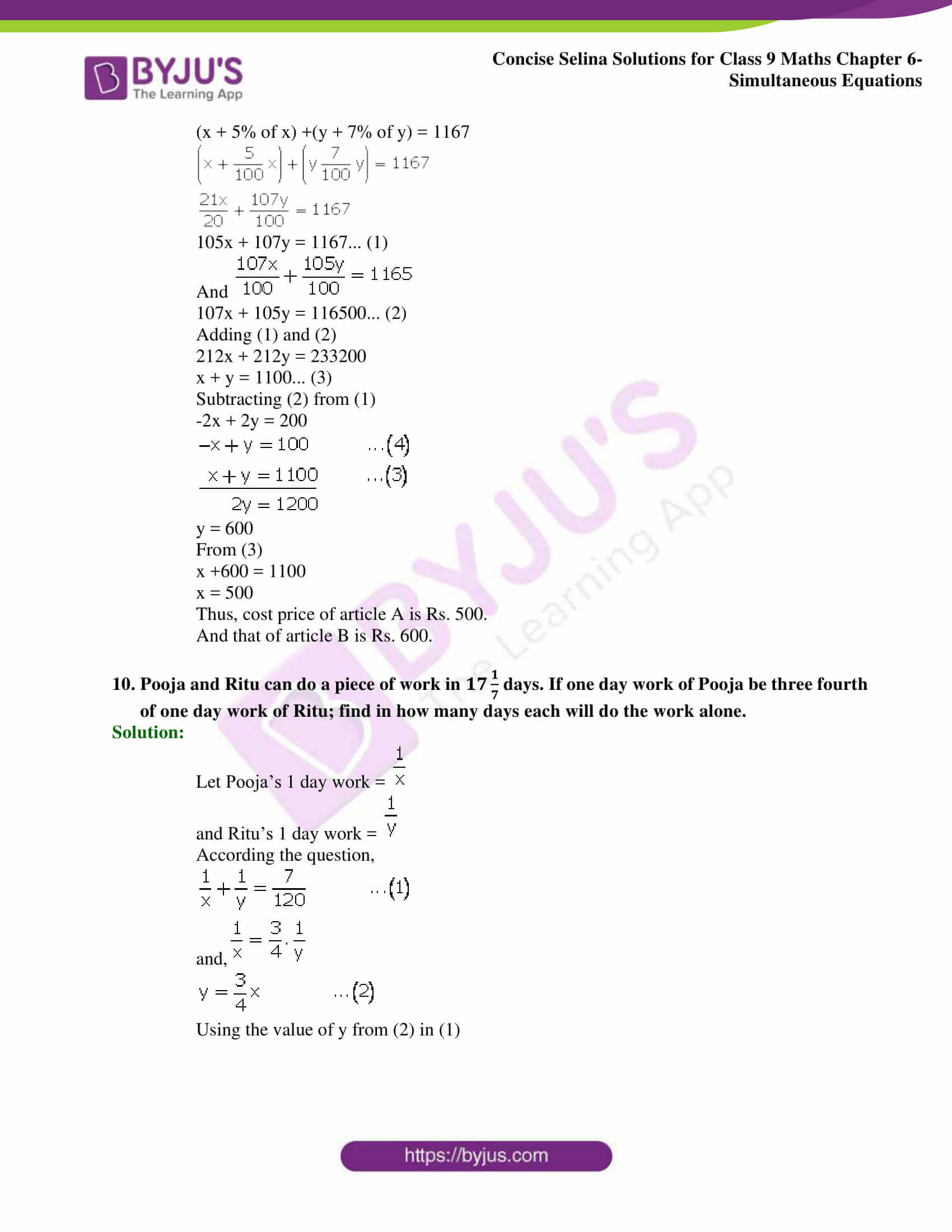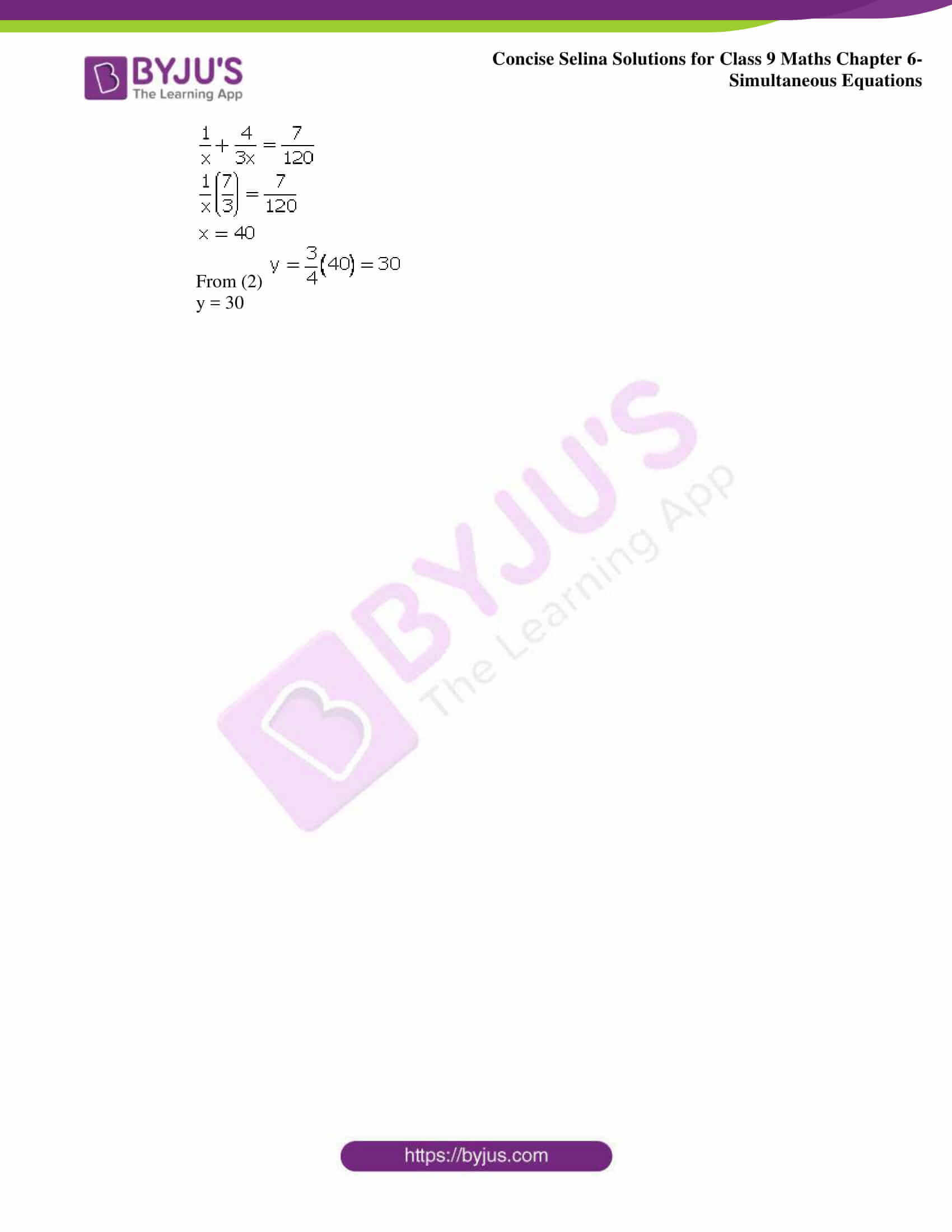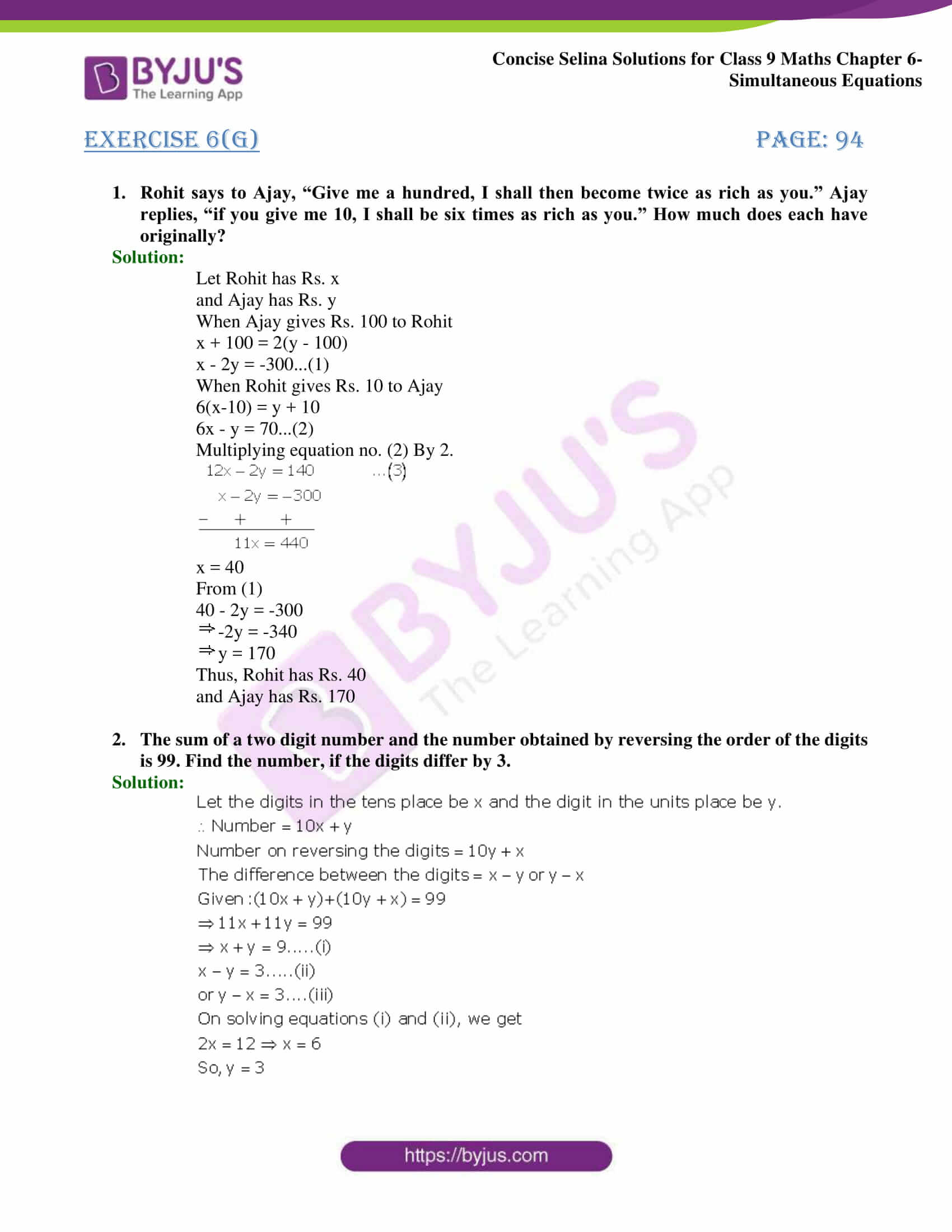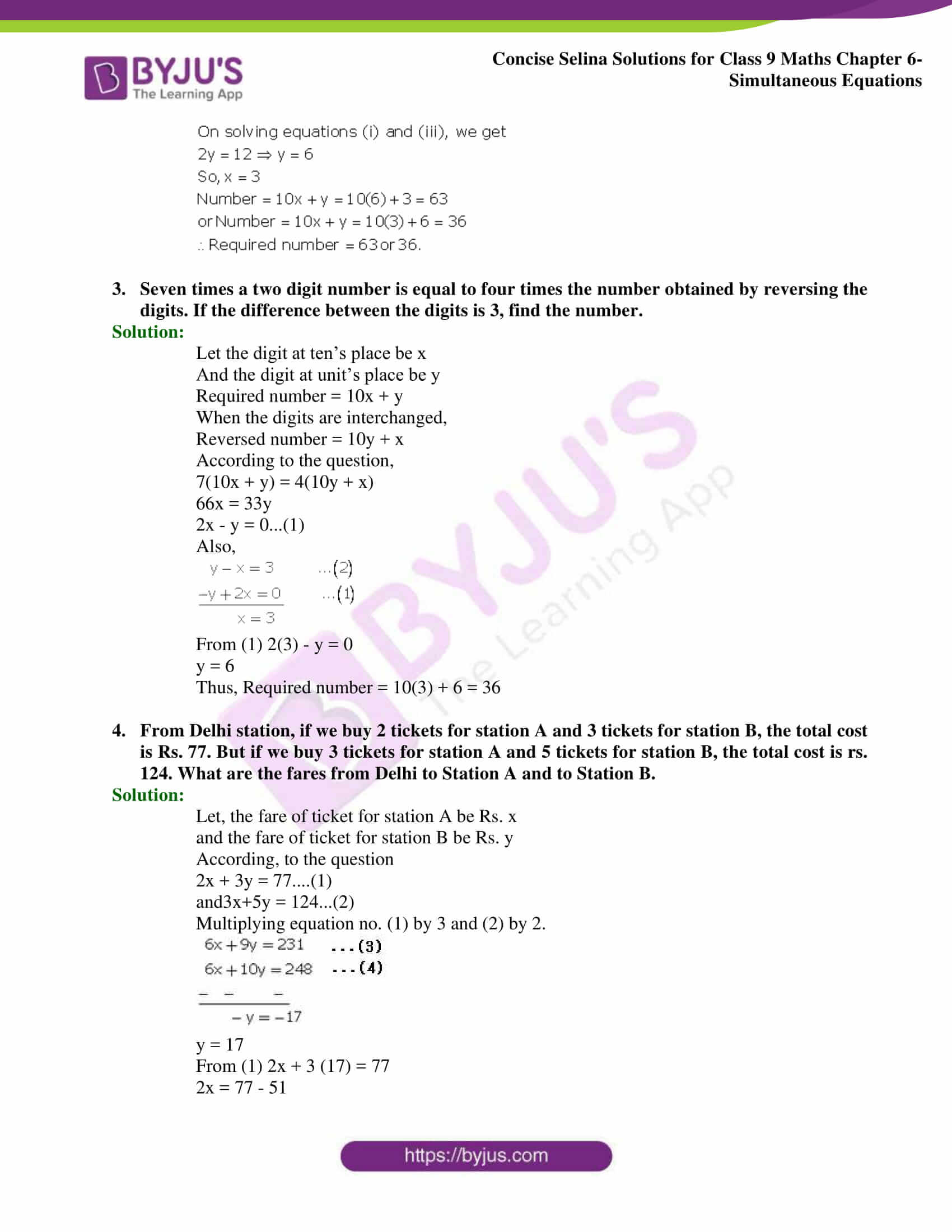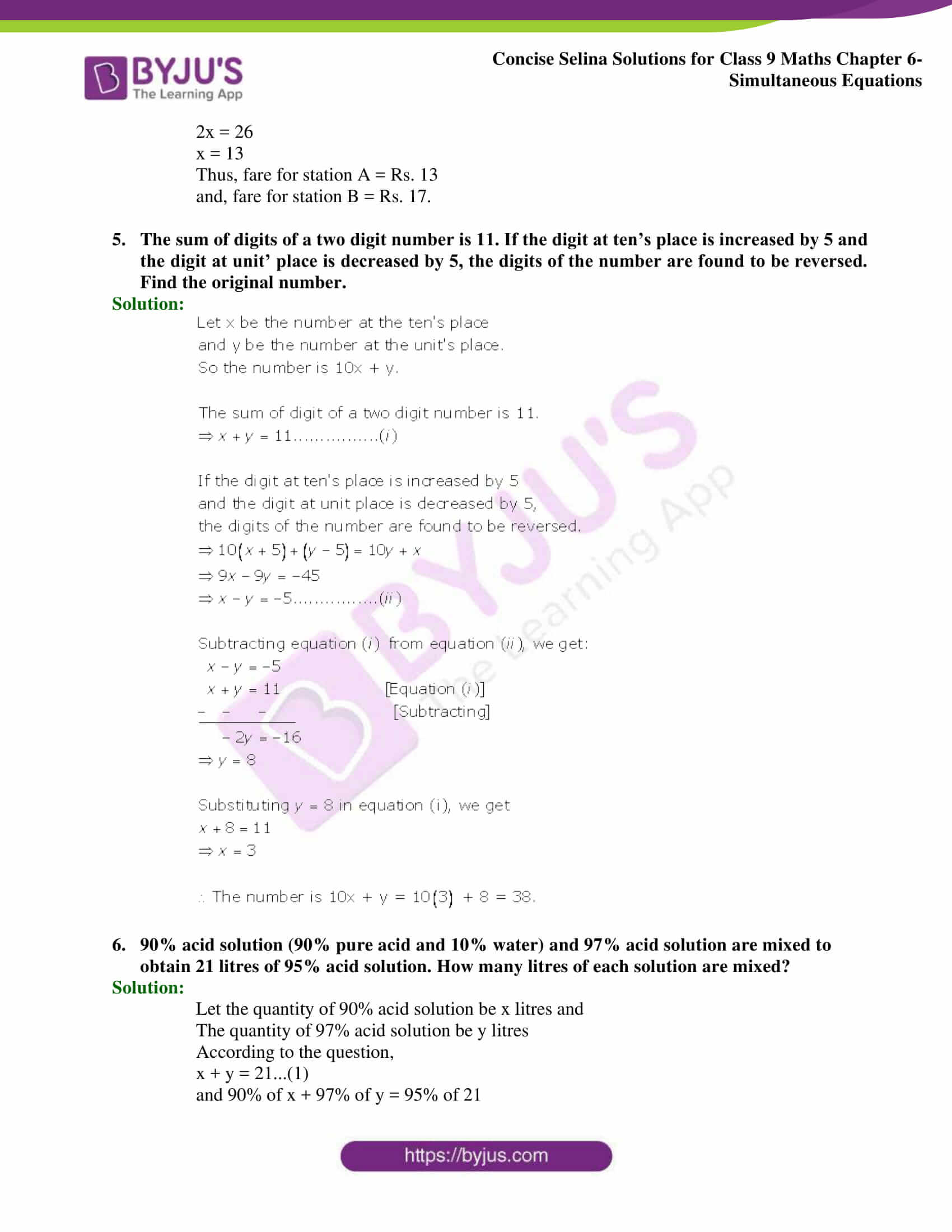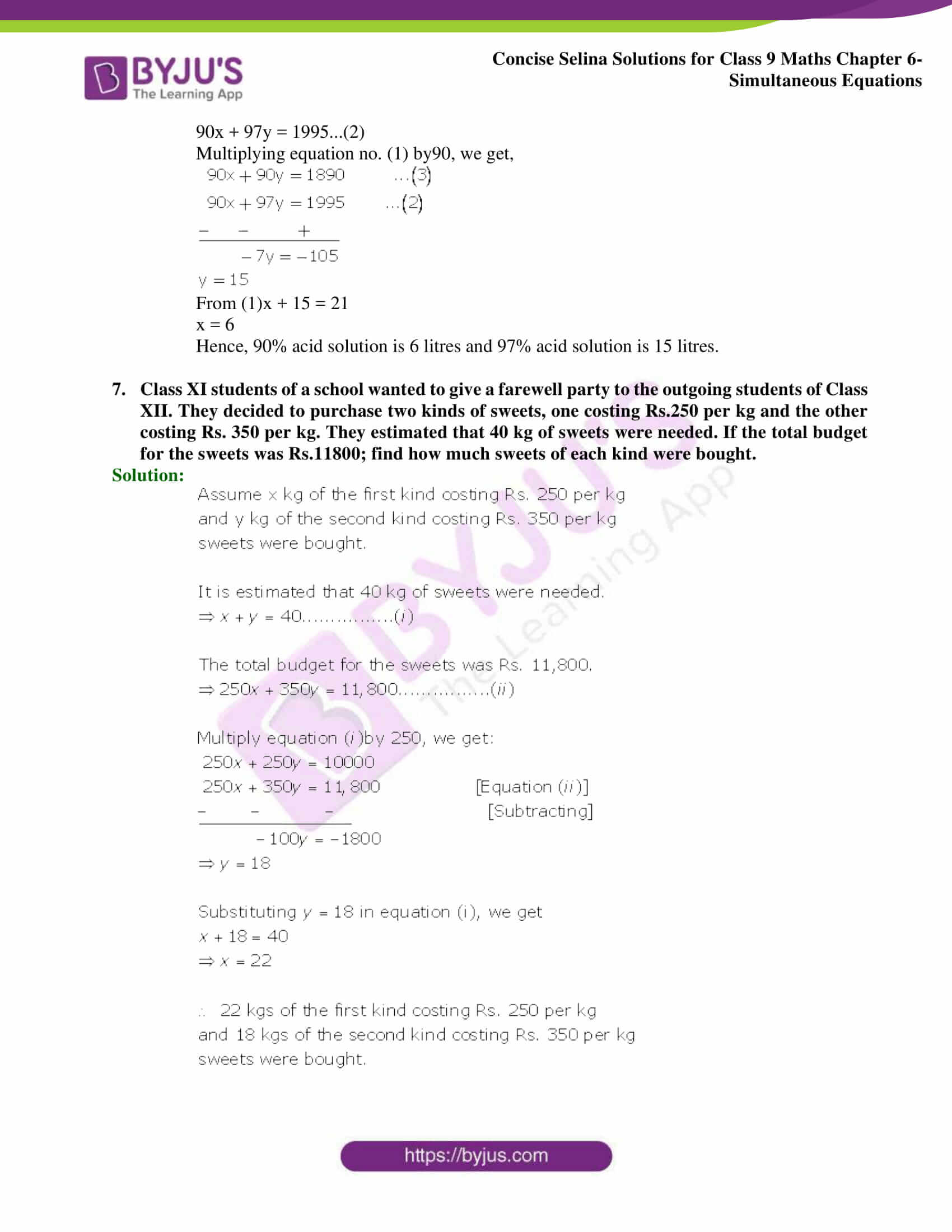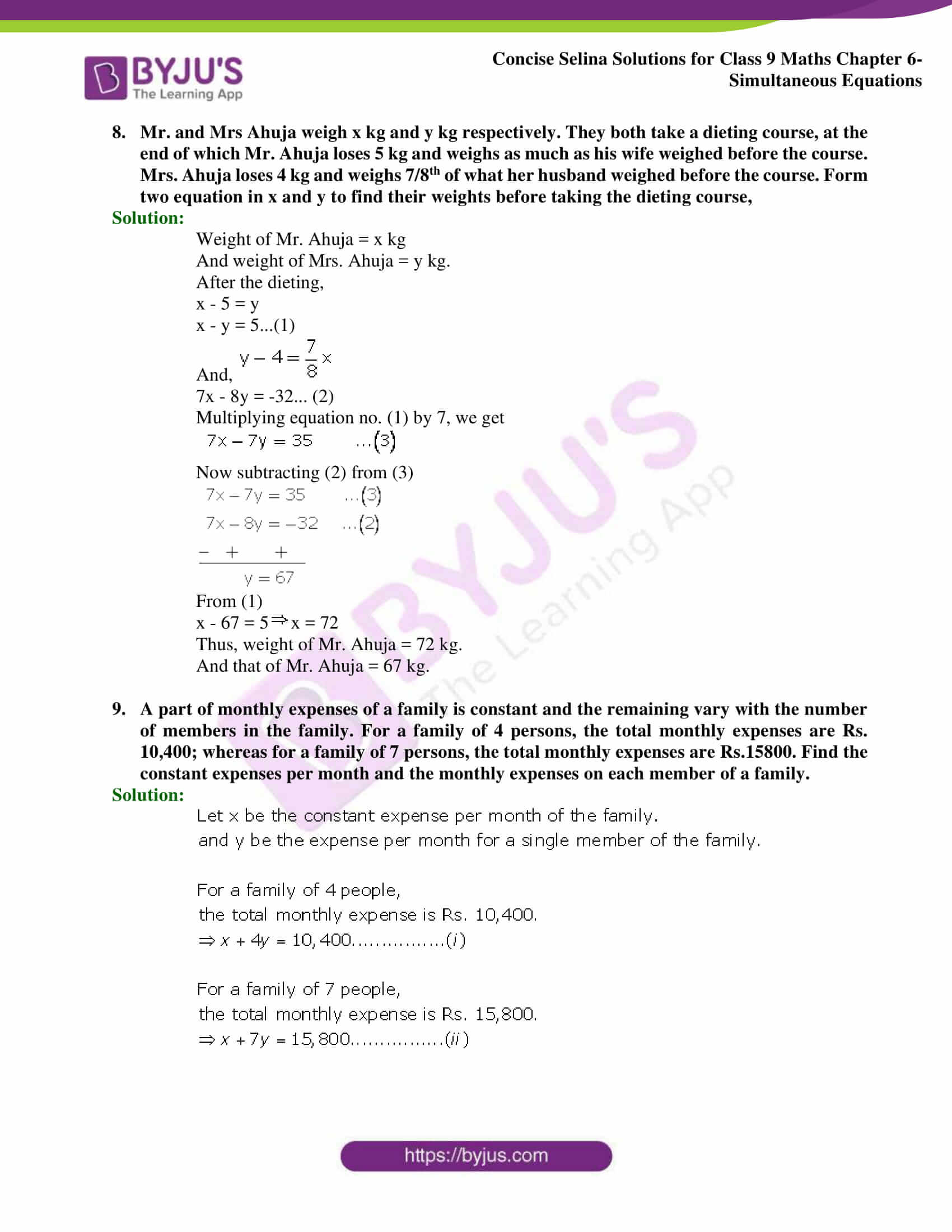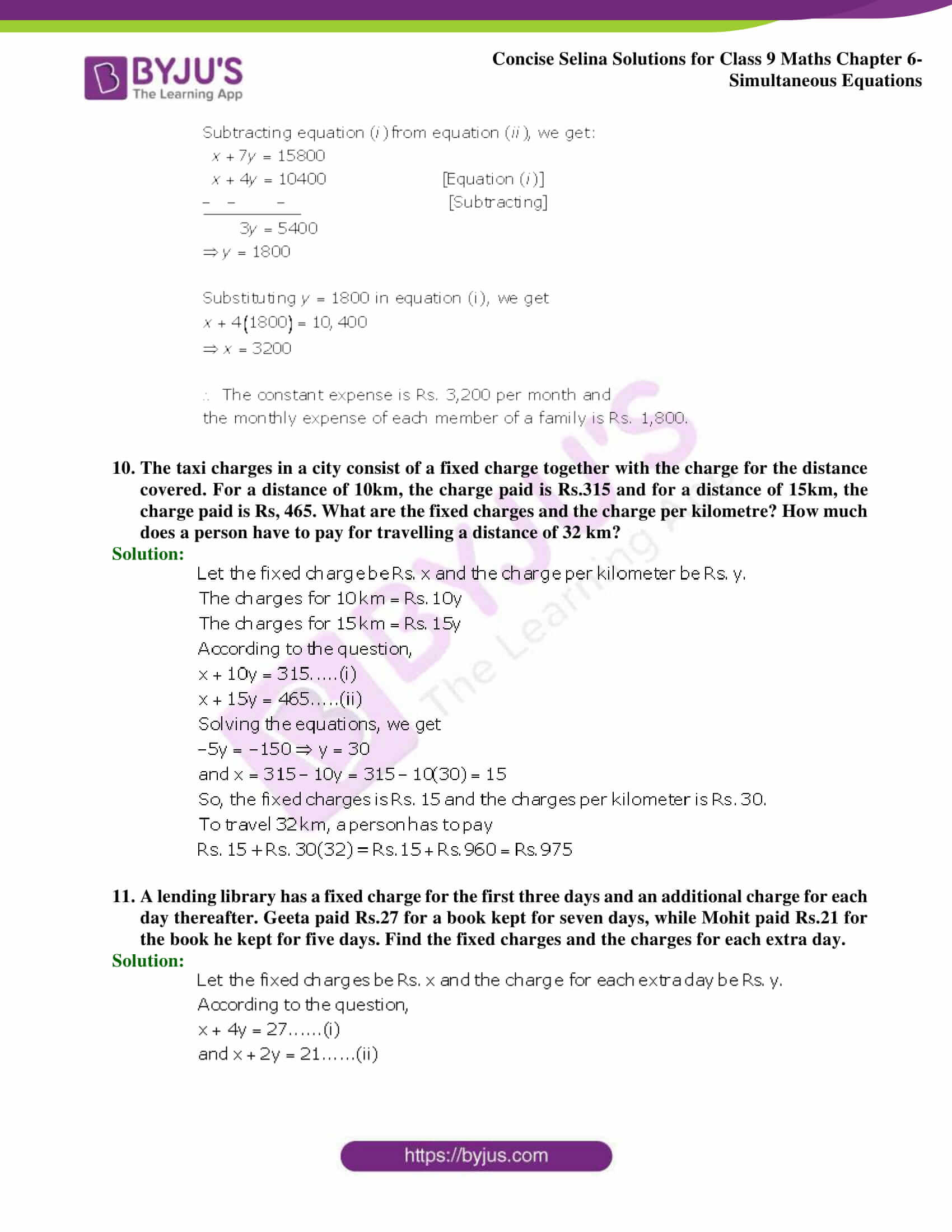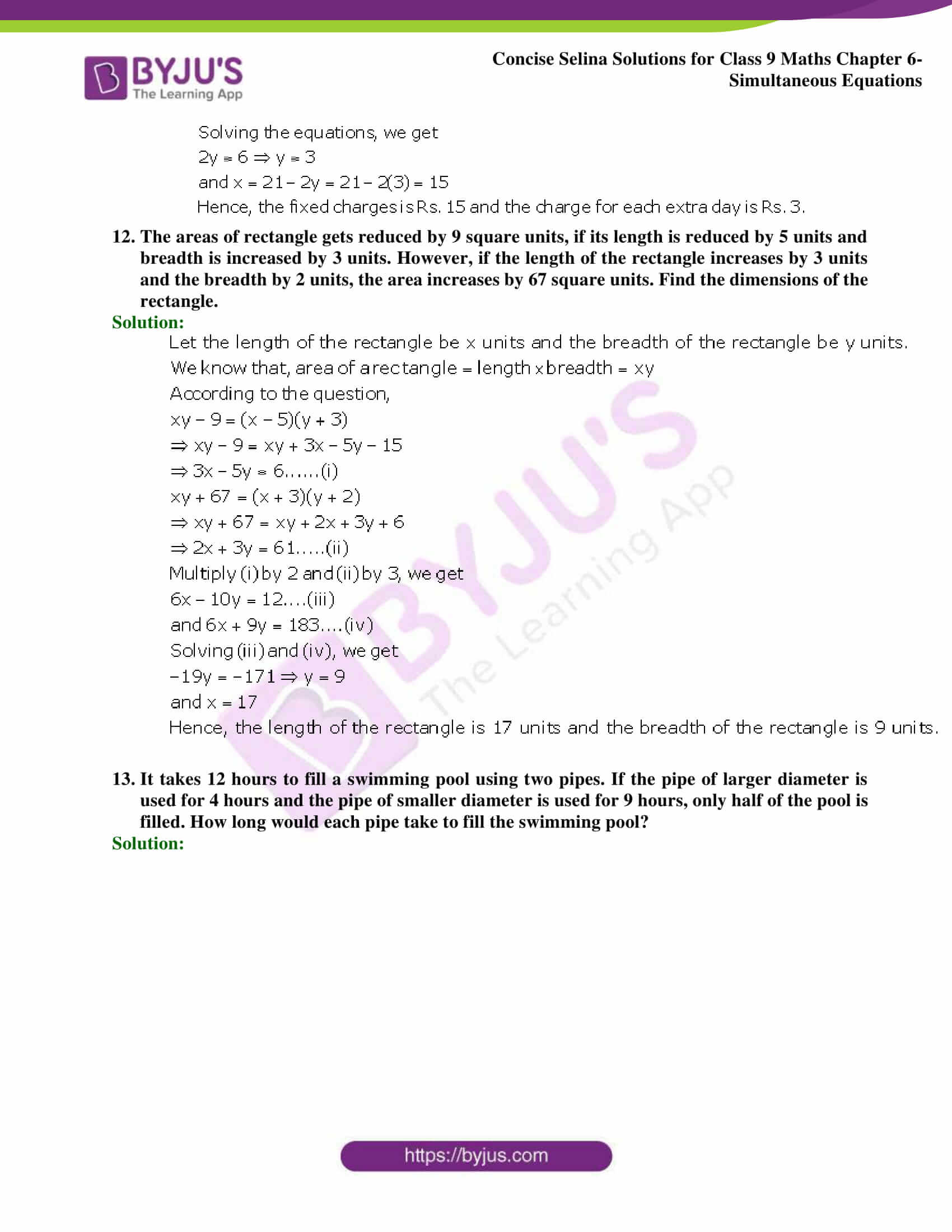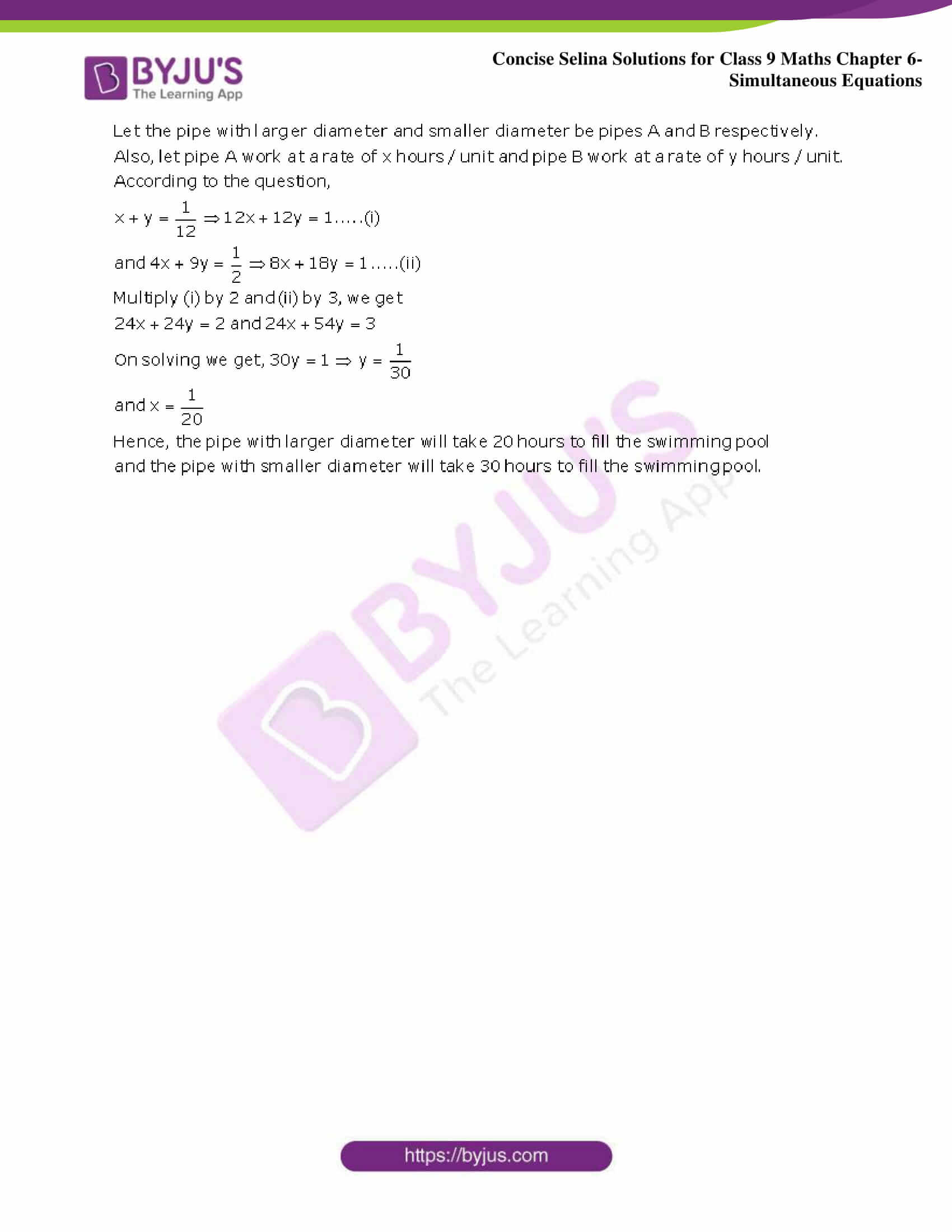## Selina Solutions for Class 9 Maths Chapter 6- Simultaneous Equations

The Chapter 6, Simultaneous Equations, contains 7 exercises and the Selina Solutions given here contains the answers for all the questions present in these exercises. Let us have a look at some of the topics that are being discussed in this chapter.

6.1 Introduction

6.2 Methods of solving simultaneous equations

6.3 Method of elimination by substitutions

6.4 Method of elimination by equating coefficients

6.5 Method of cross-multiplication

6.6 Equations reducible to linear equations

6.7 Problems based on simultaneous equations

## Selina Solutions for Class 9 Maths Chapter 6- Simultaneous Equations

An equation of the form ax+by+c=0 is called a linear equation in which a,b and c are constants (real numbers and x and y are variables each with degree 1(one). The Chapter 6 of class 9 takes the students to the depth of simultaneous linear equations in 2 variables. Read and learn the Chapter 6 of Selina textbook to learn more about Simultaneous Equations along with the concepts covered in it. Solve the Selina Solutions for Class 9 effectively to score high in the examination.